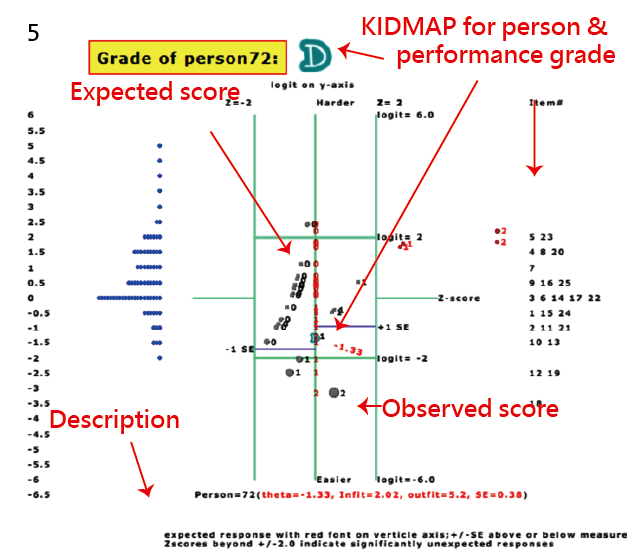## Data Types(Dichotomous response, No missing)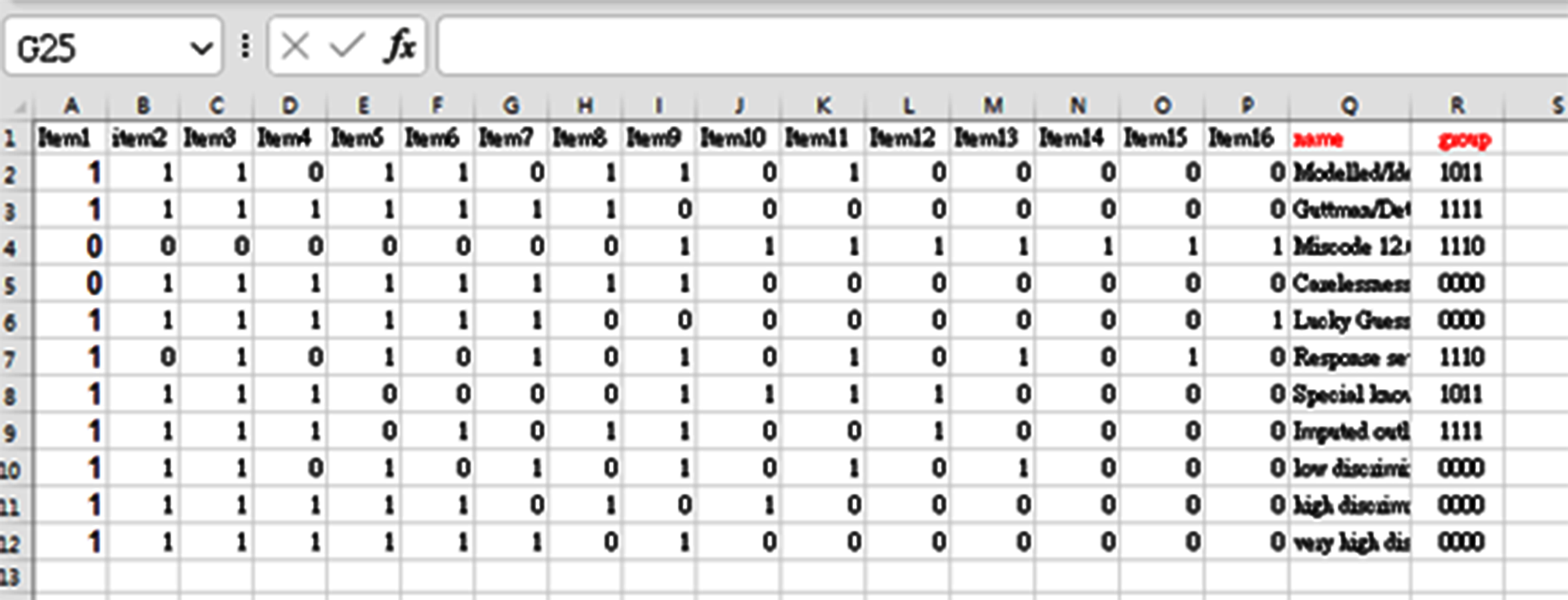## Data Types(Dichotomous response with missing rsponses using dot symbols)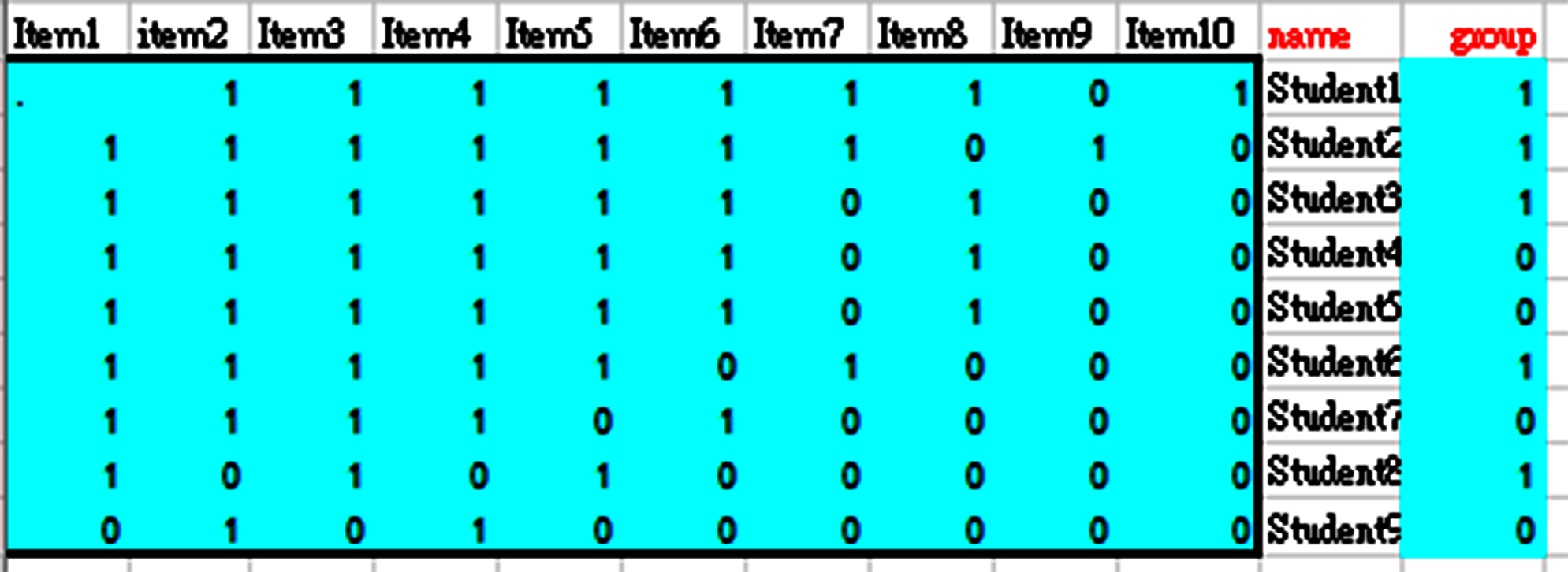## Data Types(with labels of name and group necessary in head titles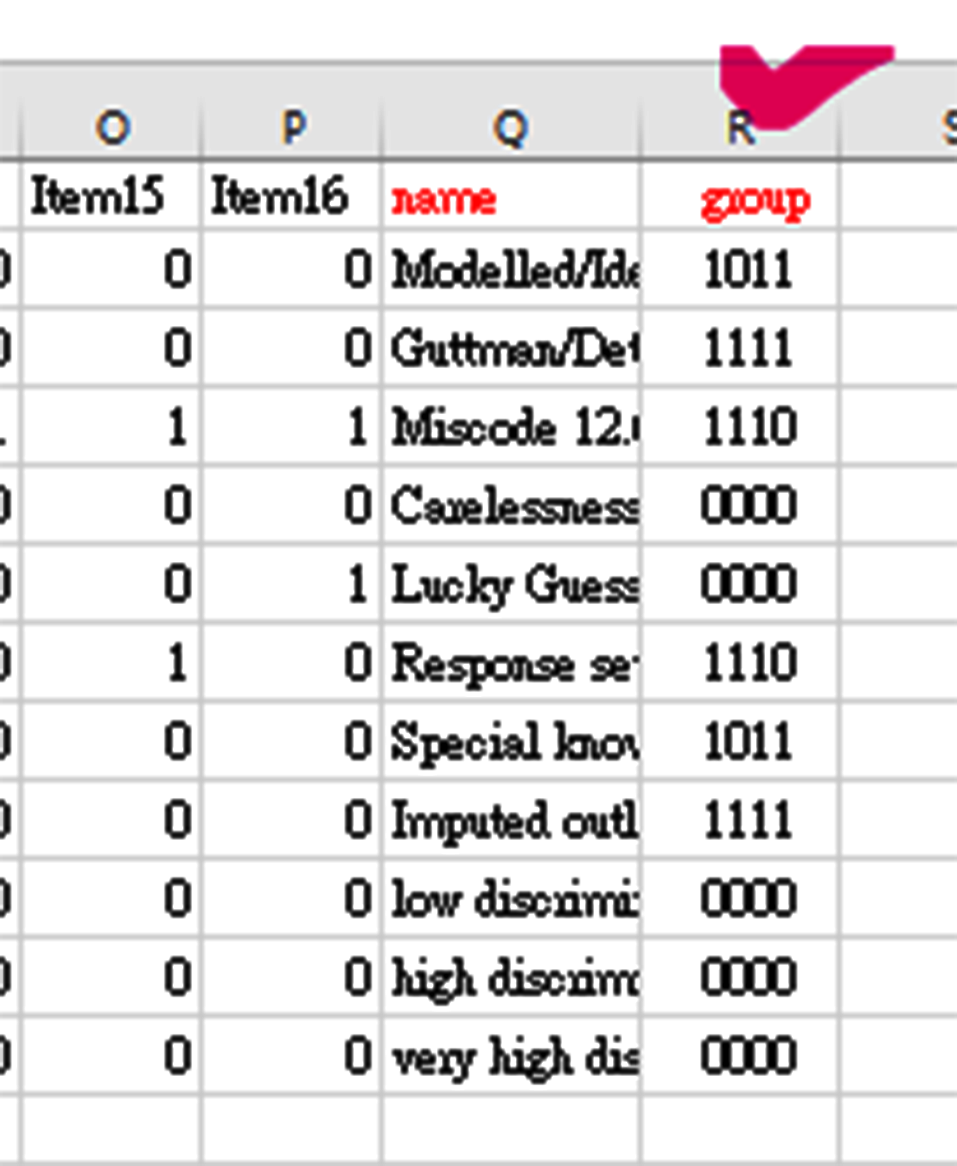## Data Types(Politomous responses)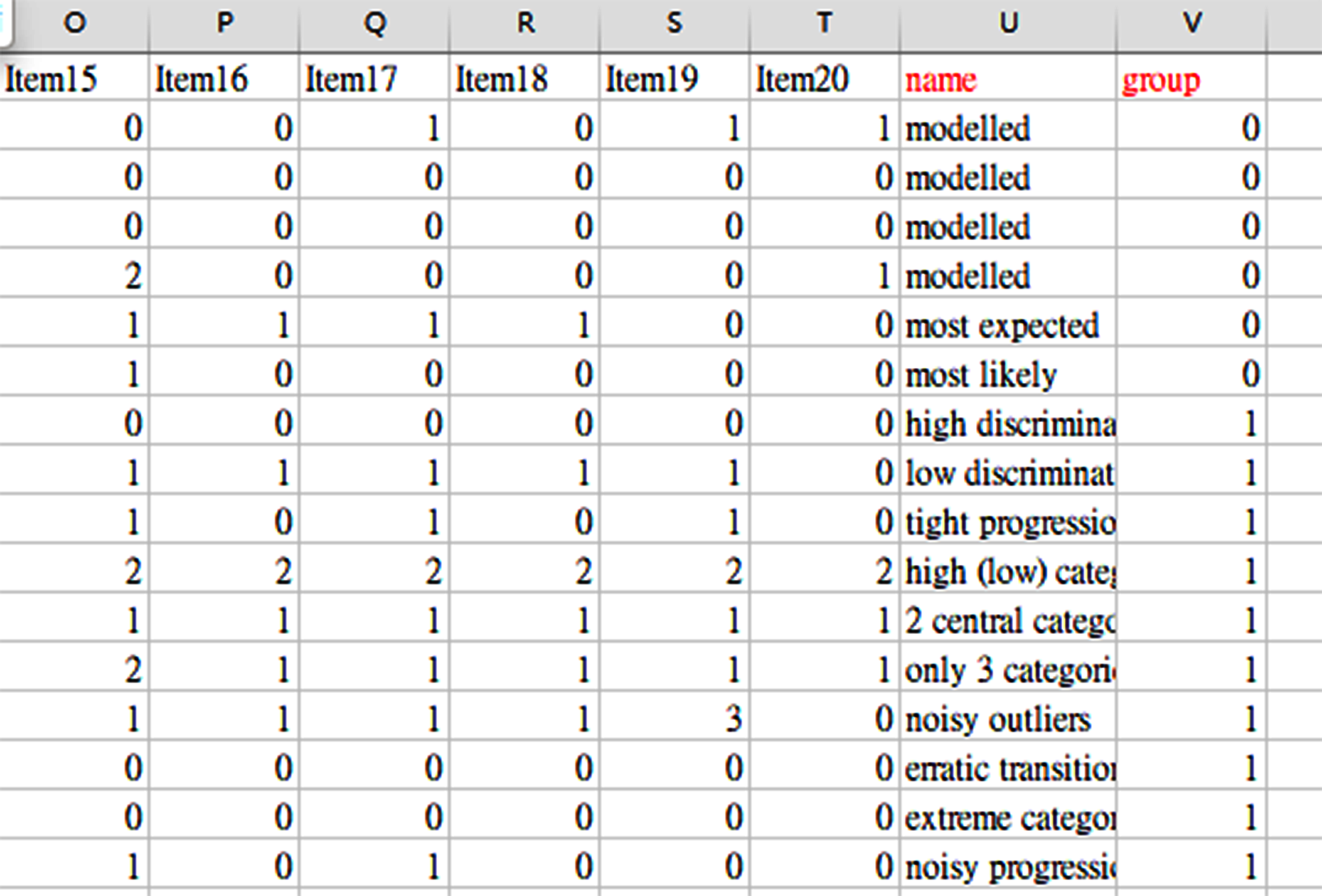## Data Entry with copy & paste approachs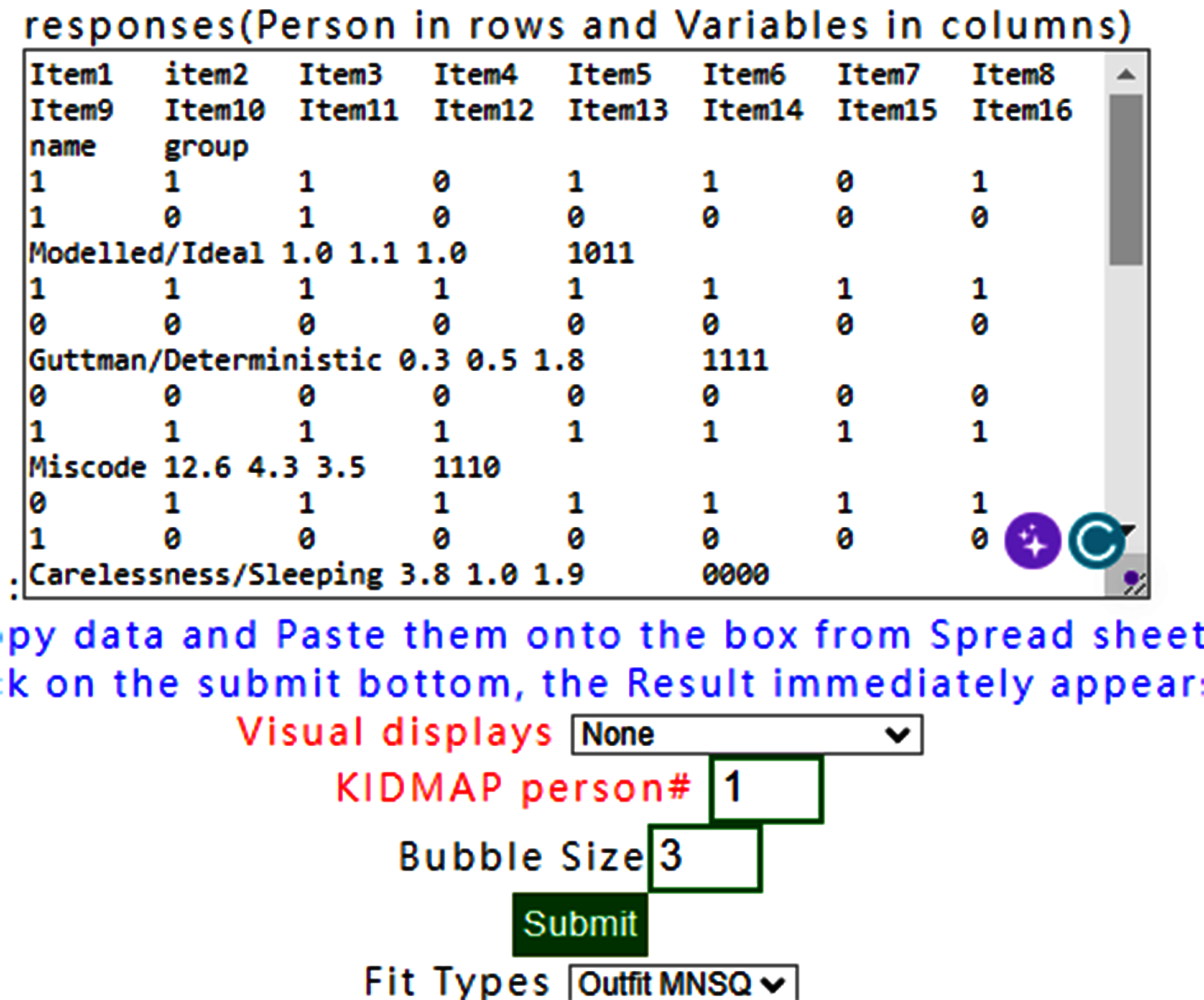## preparedness of data in two formats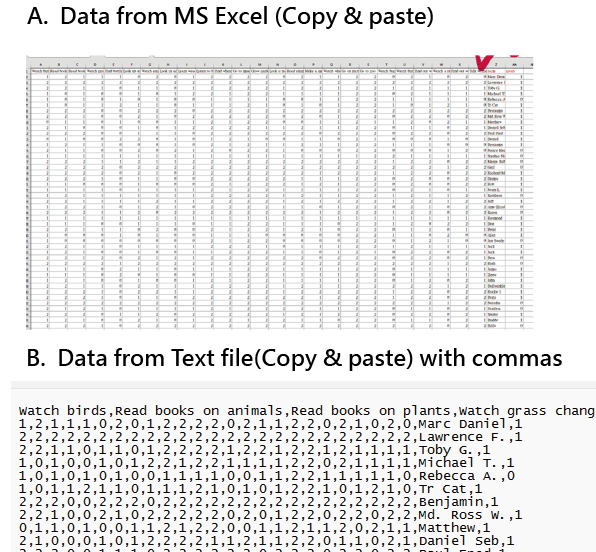## Overall data presentations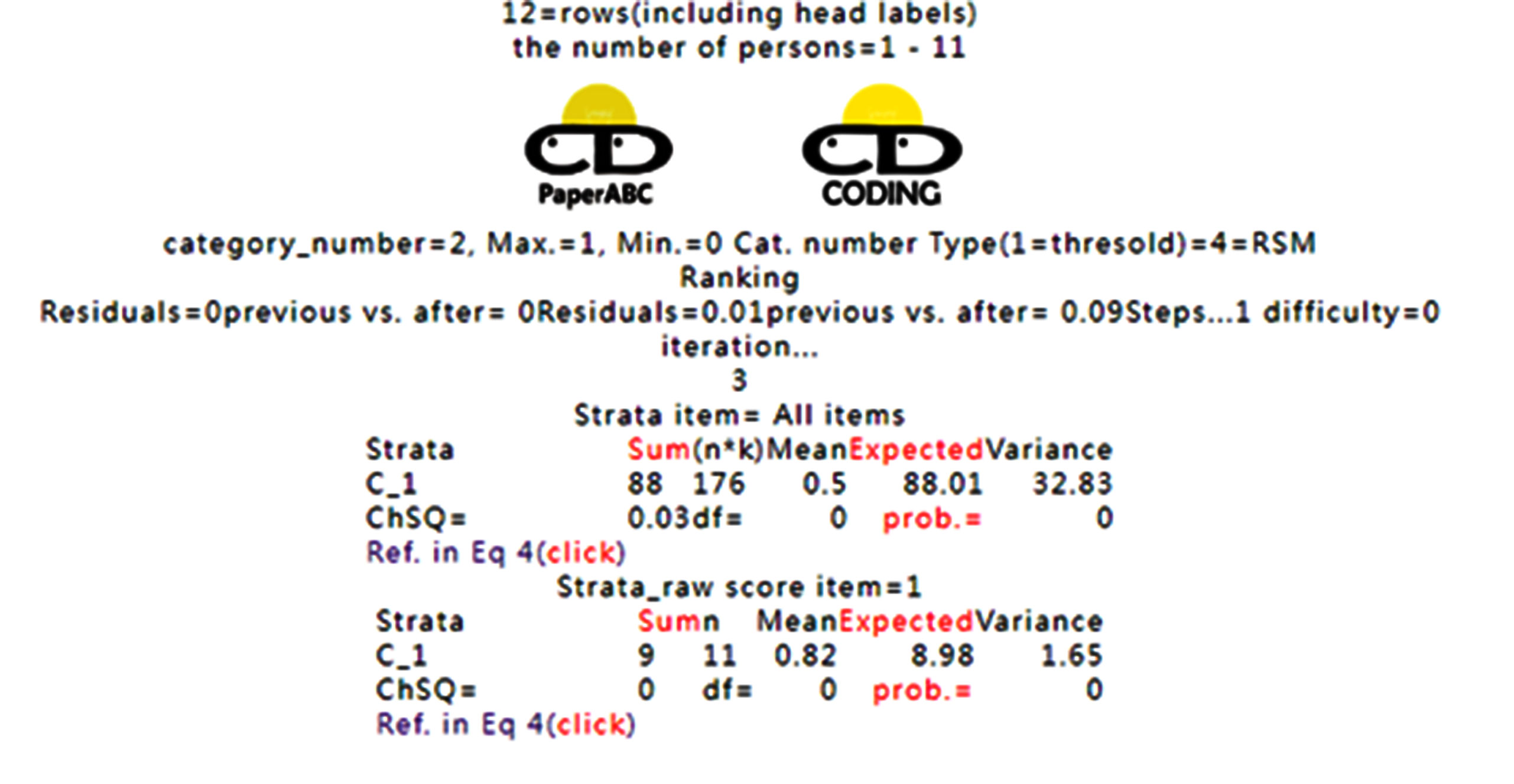## Total rows=12, including head labels. The maximum response=1 and the person number=11. Category number=2(i.e., 0 and 1): maximum=1, and minimum =0. The type of model is based on RSM(rating scale model). Model residuals are ended at the deviation between previous and after residual smaller than a criterion of 0.05. The step threshold =1(i.e. categories of 0 and 1). The step difficulty=0. By dividing strata into five strata by logit scores (e.g., >2,0.5,-0.5,2), there are one stratum only in this dataset with a summation score of 88. The model data fit cannot be computed due to df=0(see the reference at https://jsdajournal.springeropen.com/articles/10.1186/s40488-020-00108-7 with Equation 4). The counts in the stratum of C_1 are 175, which is computed by df*k, where k is the item length mentioned at the link above. On the bottom, we see the model data for item# 1 which is assigned at the previous conformation step. The model data fit is obtained via the method shown in the table. Notes that the strata are computed and assigned the labels as programs below: kidat=round((6-person(jk))*2,0) ‘ person(jk)=person measure if kidat<0 then kidat=0 if kidat>25 then kidat=25 ranking=Fix(kidat/5)+1 alpheta="ABCDEFGJIJK" remainer=kidat mod 5 rk=mid("ABCDEFGJIJK",ranking,1) ' & right("0" & (remainer+1),2) personrk(jk)=rk ‘assigning the label the person if instr(rktext, rk)=0 then rktext=rktext & rk end if cutting(ranking)=cutting(ranking) +round(person(jk),2) ‘ used to compute the mean measures in each stratum, which is used in drawing the ICC later.## Note. the Grade label is defined in the previous code mentioned in notes. Person estimations are listed above by series of number, group label, groups by measures in logit(i.e., C03 is around at Zero logit. Theta is person measure(e.g., all at zero logit). Model SE(standard error=sqrt(1/variance for person). Infit and Outfit MNSQs are followed. * indicates significantly higher than 2.0. Chisqure is the results of summation of squared Zscore(=[(obs.-exp)/SD]^2) based on chiSQ test.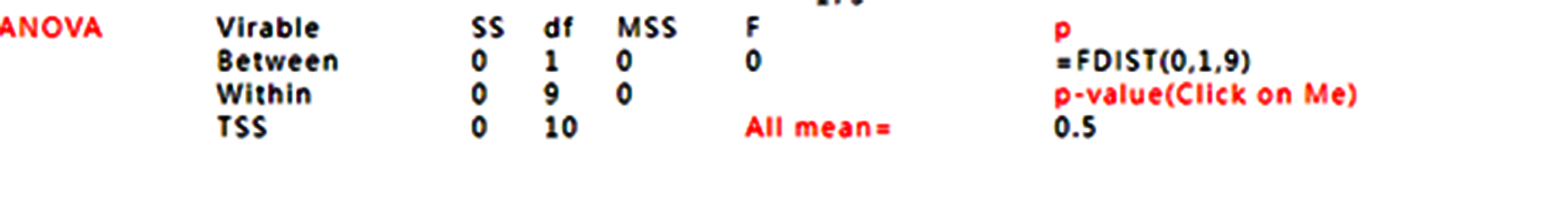## ANOVA for groups F(0,1,9) and check the probability at https://www.socscistatistics.com/pvalues/fdistribution.aspx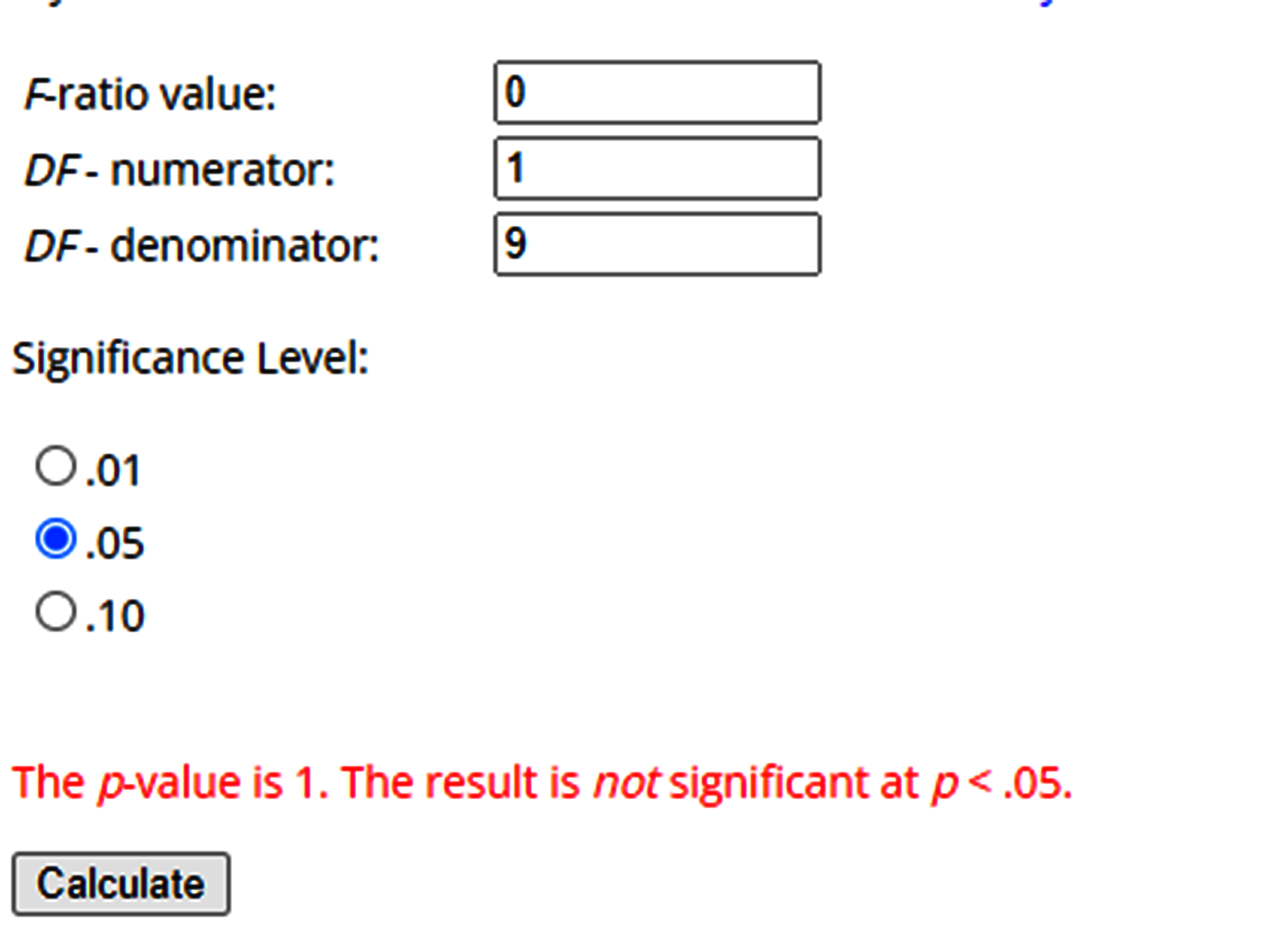## Indicating that no difference was found between the two groups.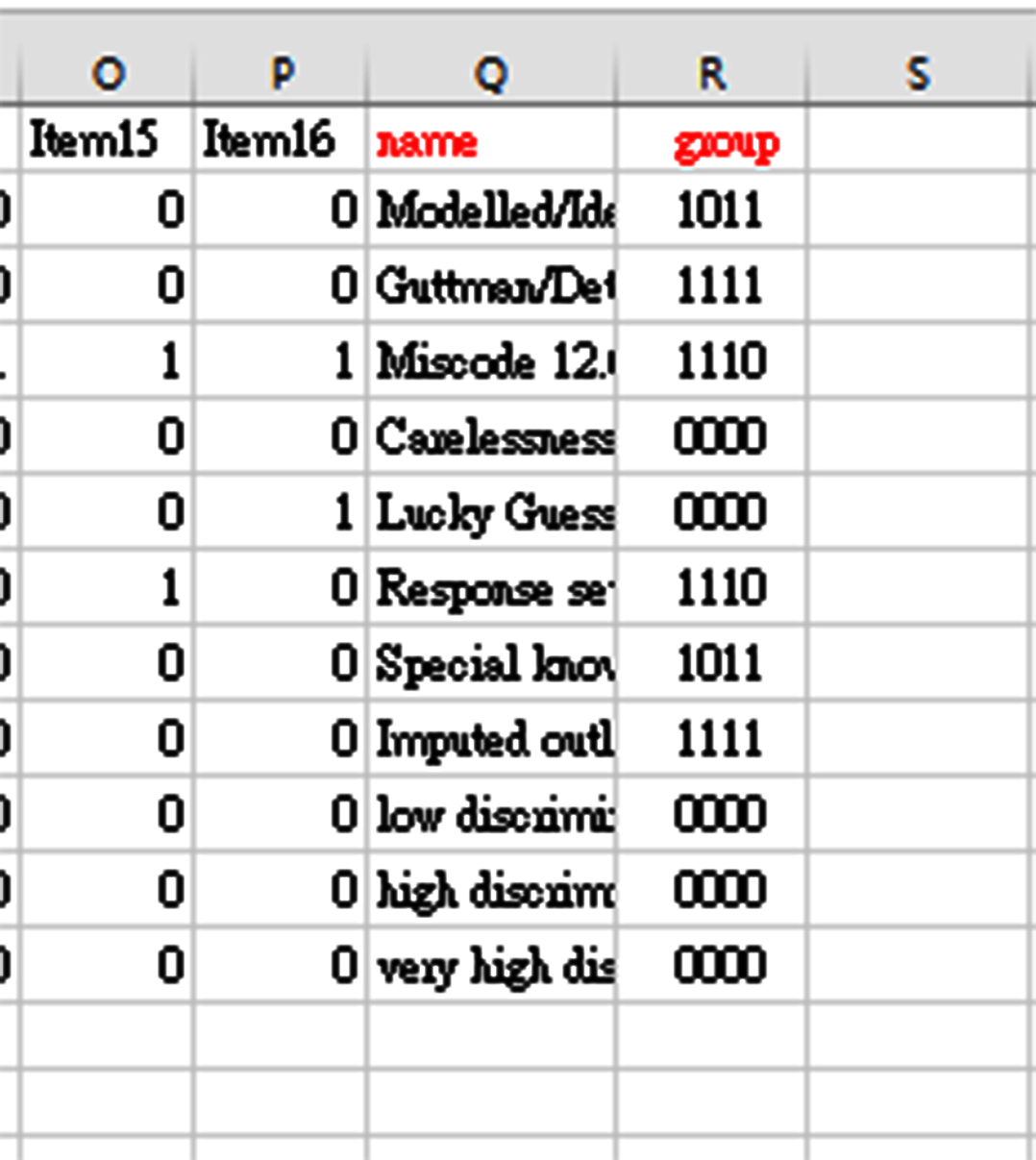## It is worth noting that the group is based on the first digit in group contents.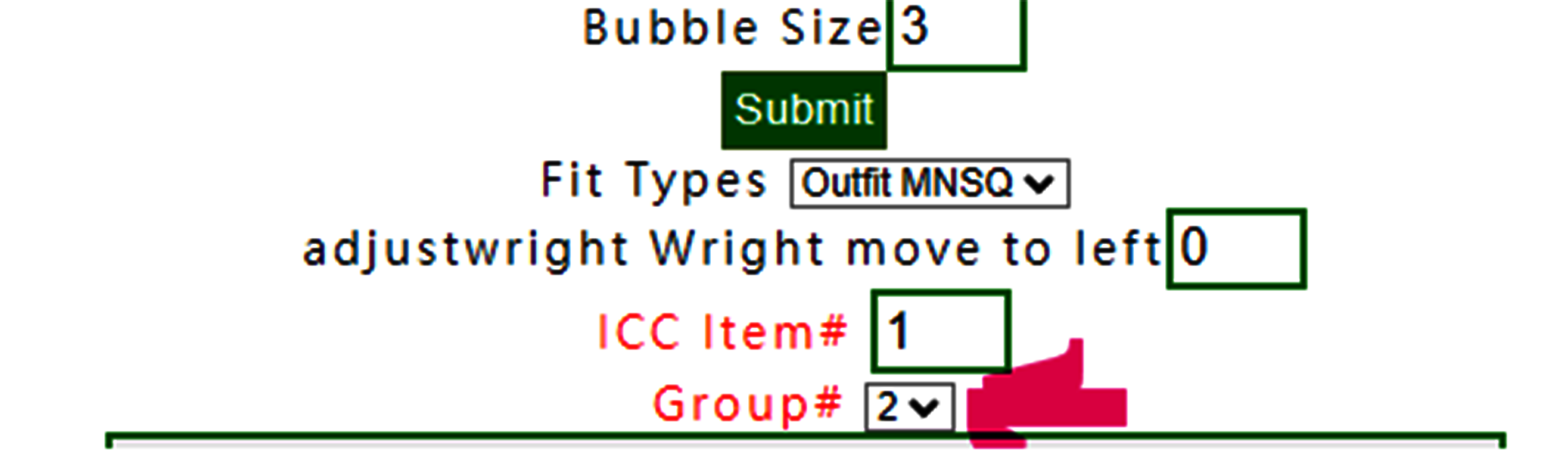## If the group label of two is selected, the ANOVA would be different:## In the F test, we observe that the two are 0.31 vs. 0 when compared to the previous group defined in the selection of the group definition in the combo box in the confirmation step.## Unexpected responses are shown in black and red bubbles, indicating under expectation(in black) and over expectation(in red), ie. Zscore=(obv. – exp.)/SD>2.0=over expectation and <-2.0 indicating under expectation.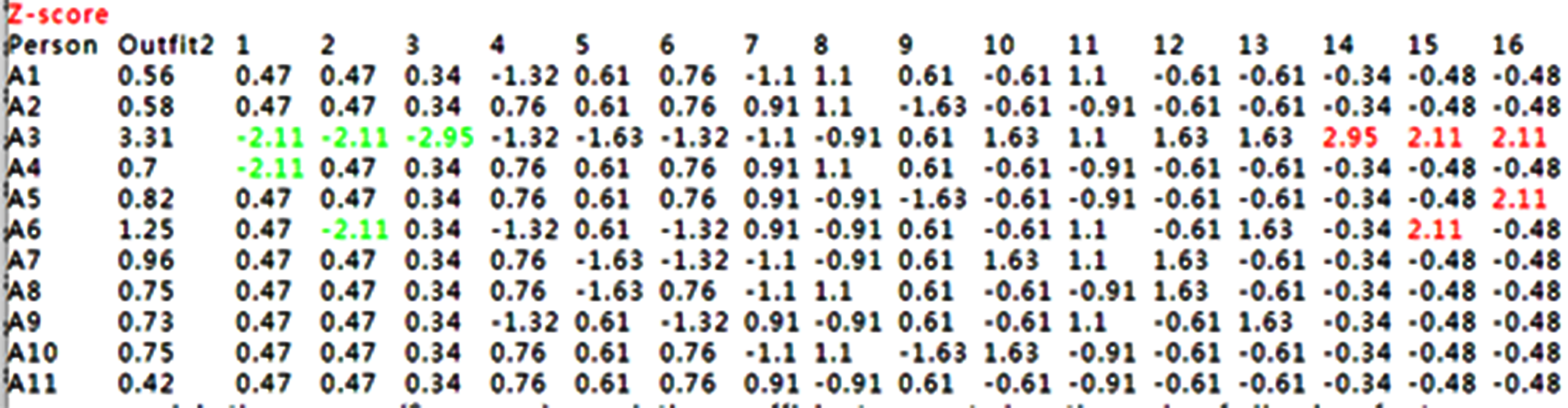## Unexpected responses are shown in Zscores in green and red font.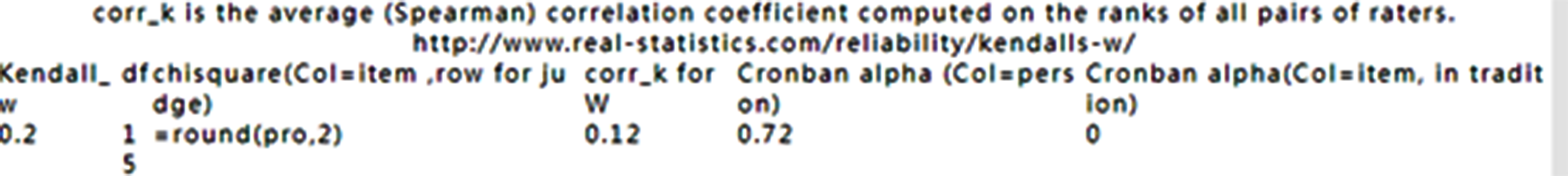## Cronban’s alph for items and persons in 0.72 and 0. Respectively. The Kendall coefficient refers to https://www.real-statistics.com/correlation/kendalls-tau-correlation/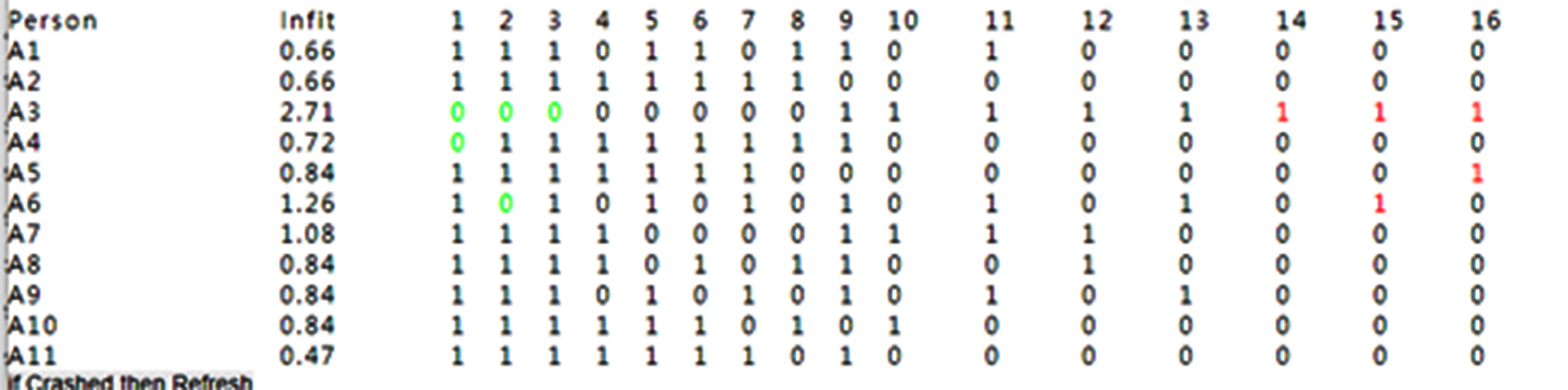## Unexpected response with original data## Item difficulties are listed with measures, SE, Infit and Outfit MNSQs. The correlation coefficient between raw scores and item difficulties is negative. A high raw score has a lower item difficulty.## 2.Summary Table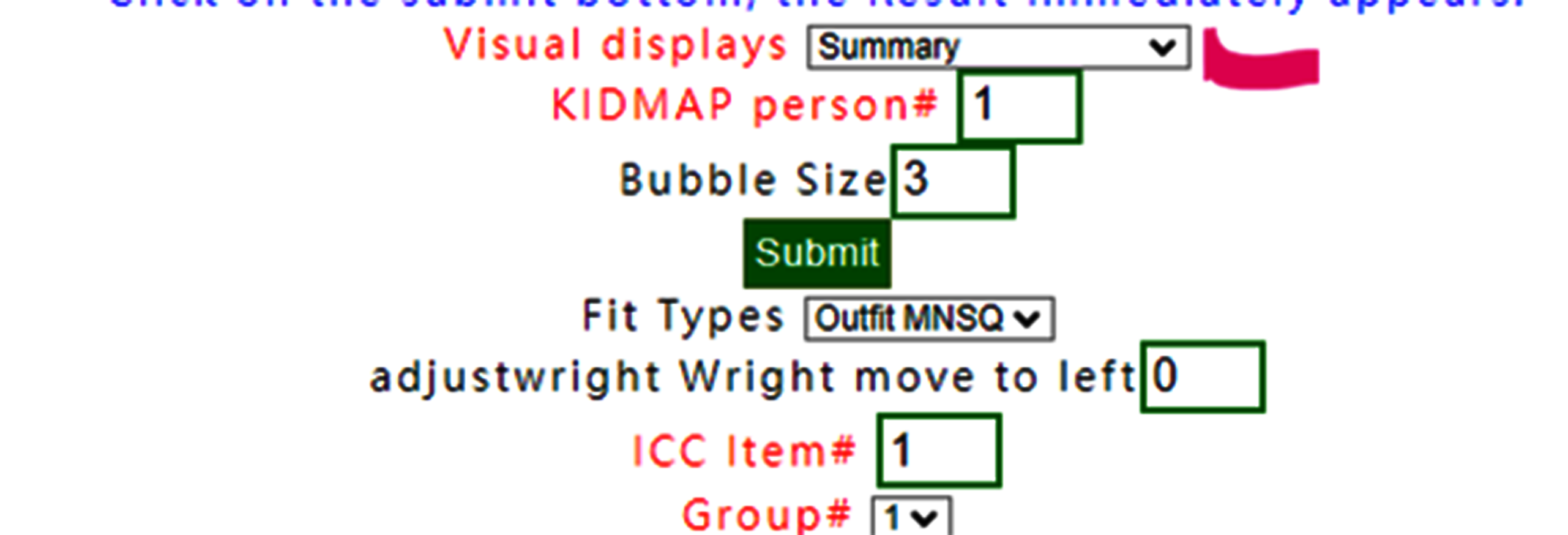## 2.Summary Table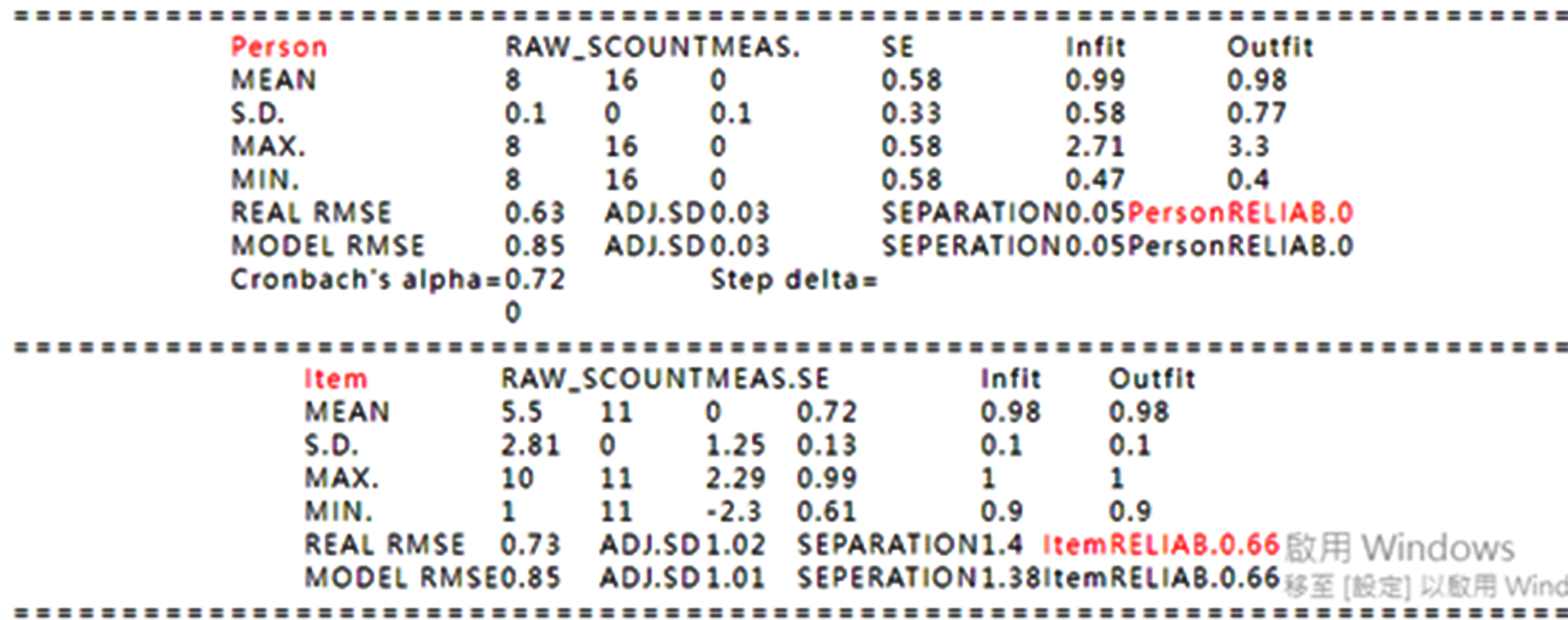## 3.Overall Fit Stat.## 2.Summary Table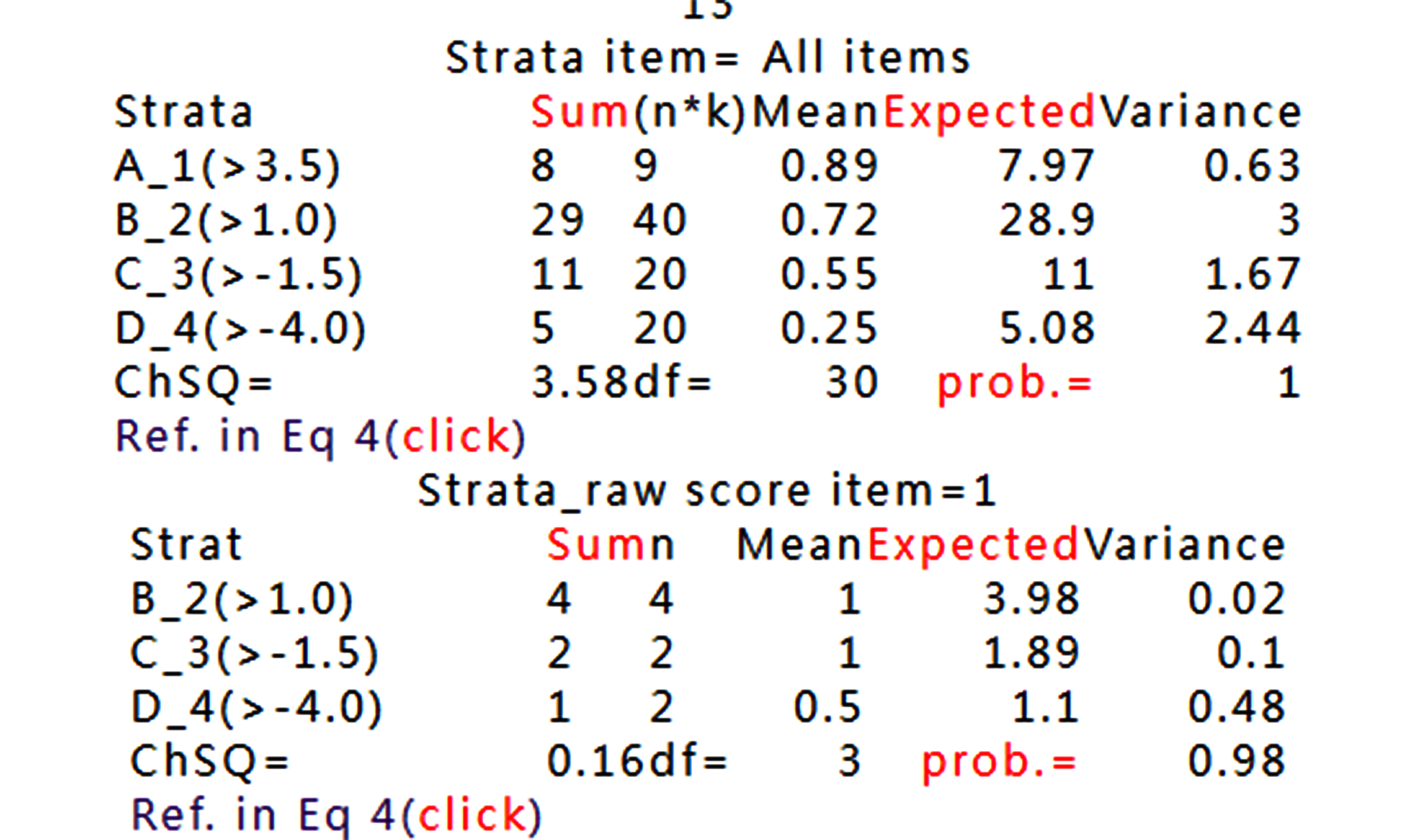## 4.ANOVA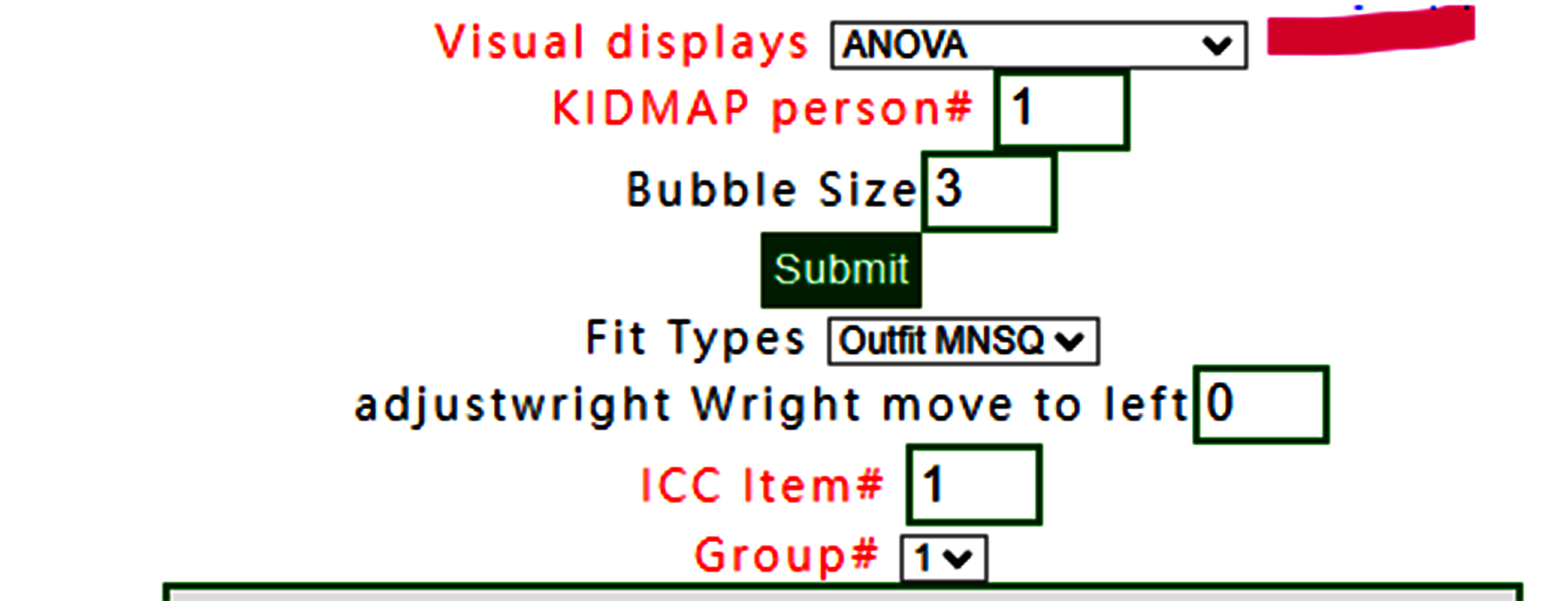## 2.Summary Table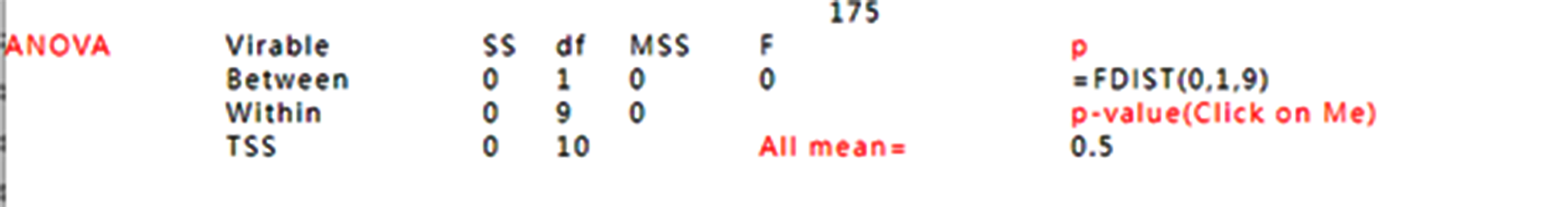## 5.Wright Map## select Wright Map.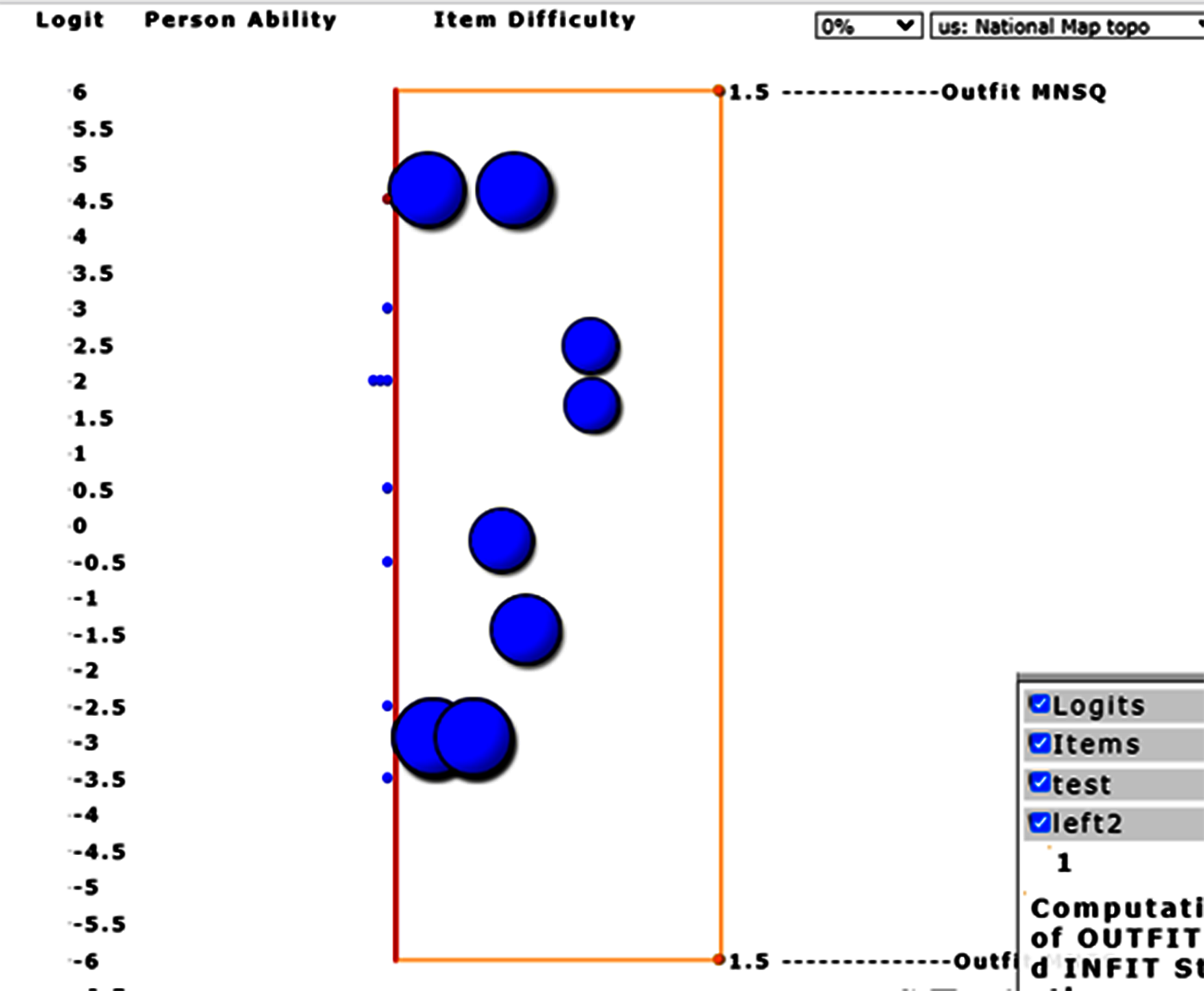## ToTodisplay Wright Map.## To adjust bubble size to 1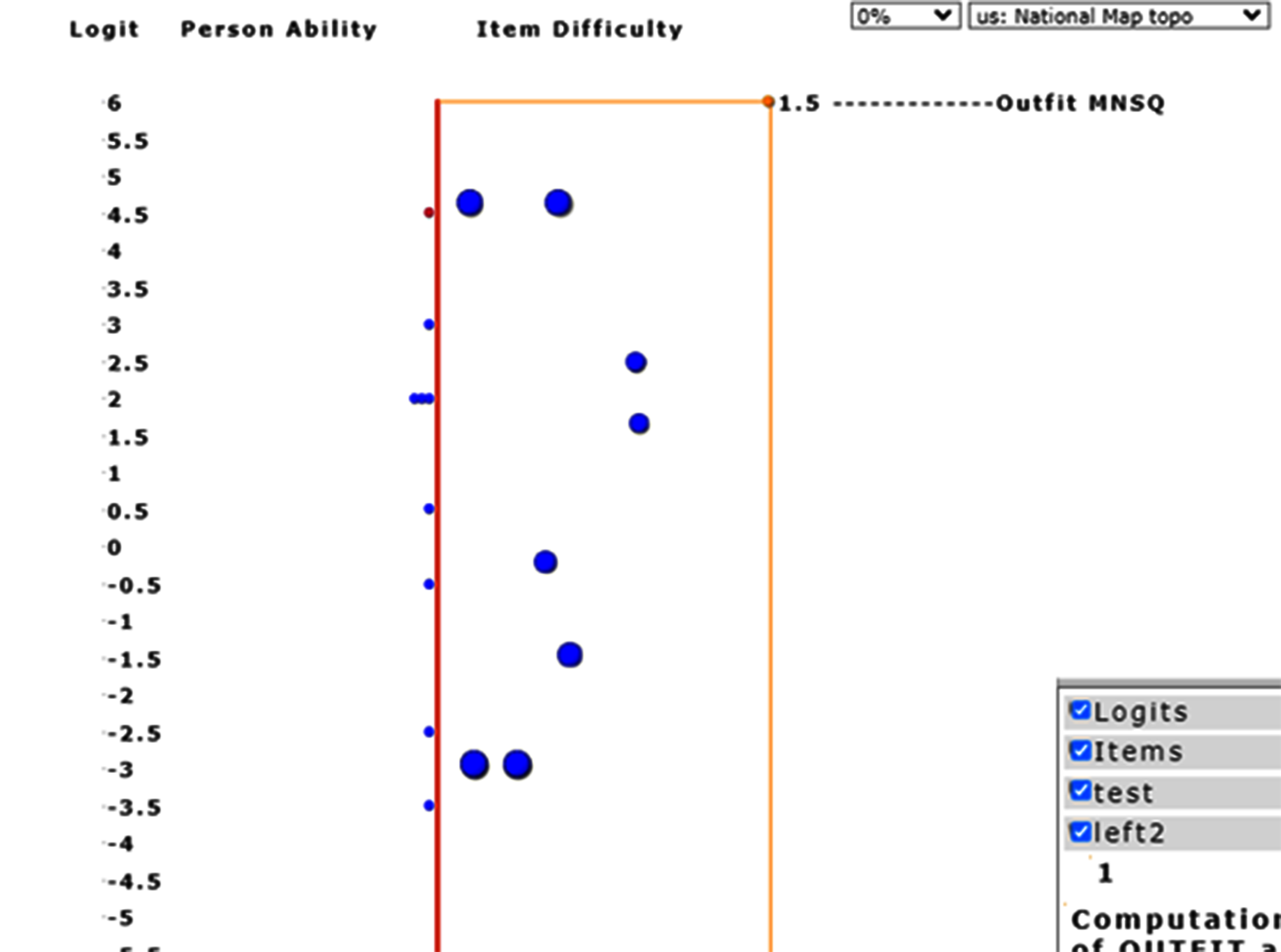## Bubbles are adjusted to an appropriate layout.## To adjust the fit statistics with infit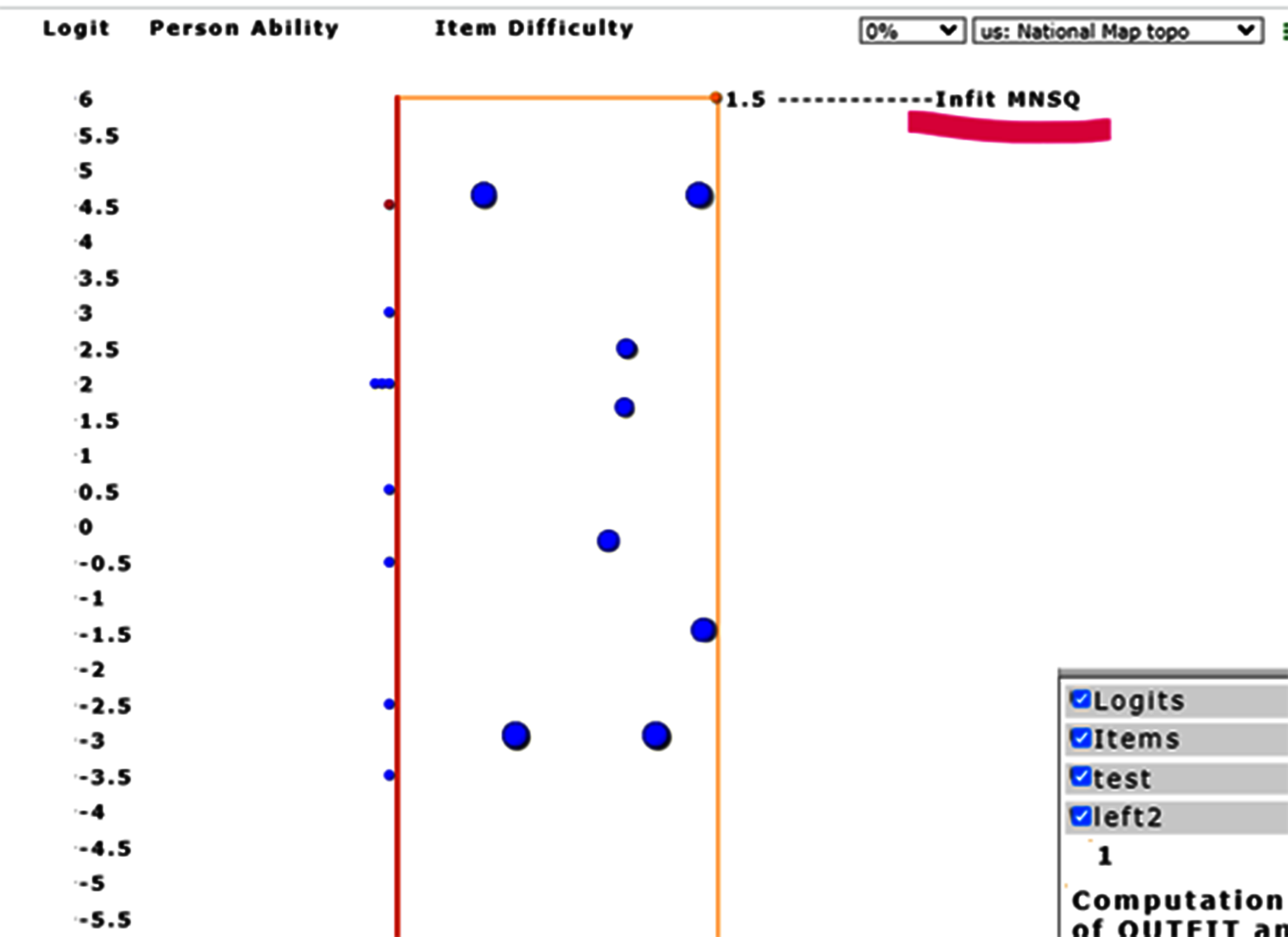## Item infit MNSQs are displayed, different from those with Outfit MNSQ in the previous example.## If dash is selected.## the results are identical due to no step difficulties in the dichotomous scale as shown below:.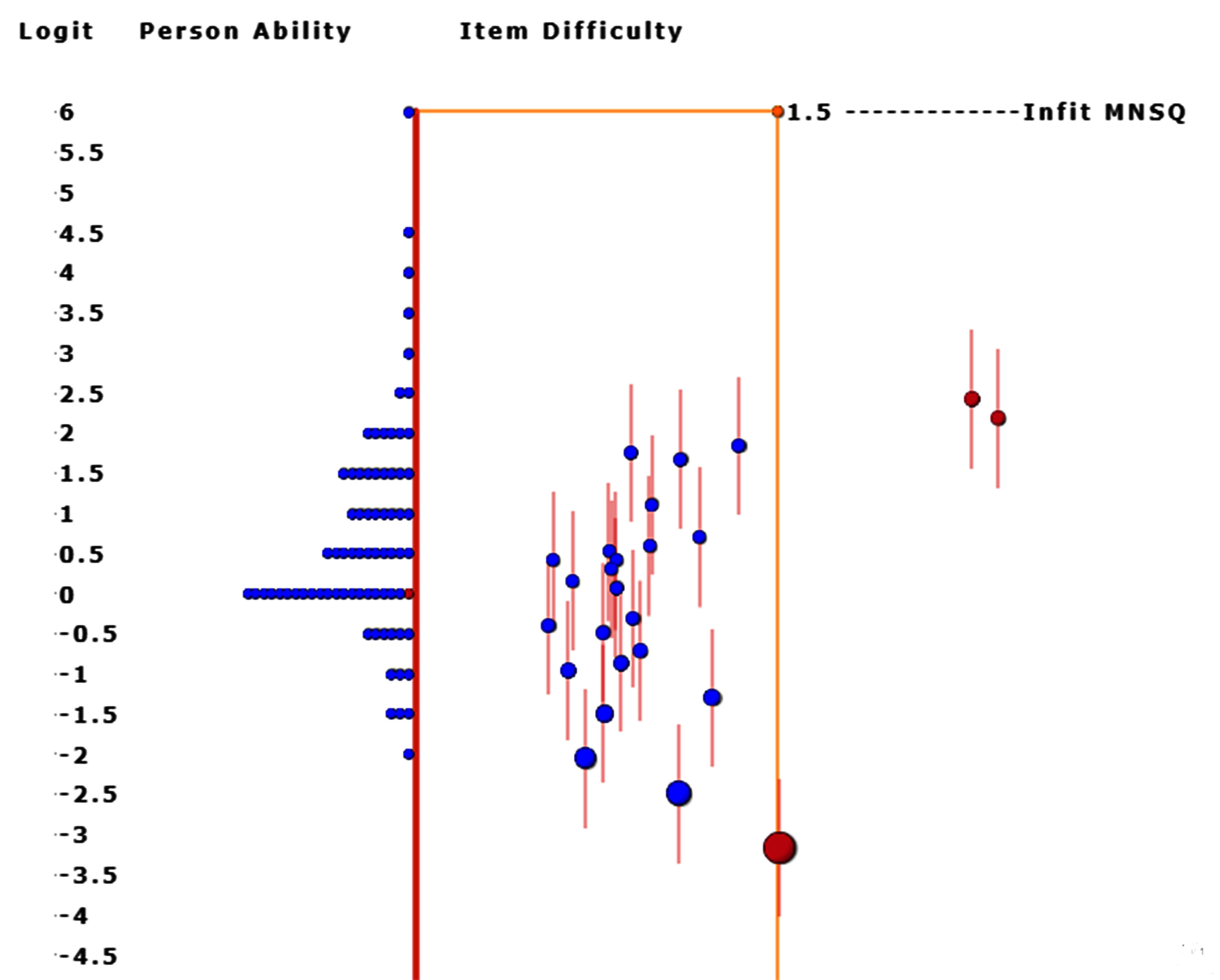## 6.Wright Map(Groups)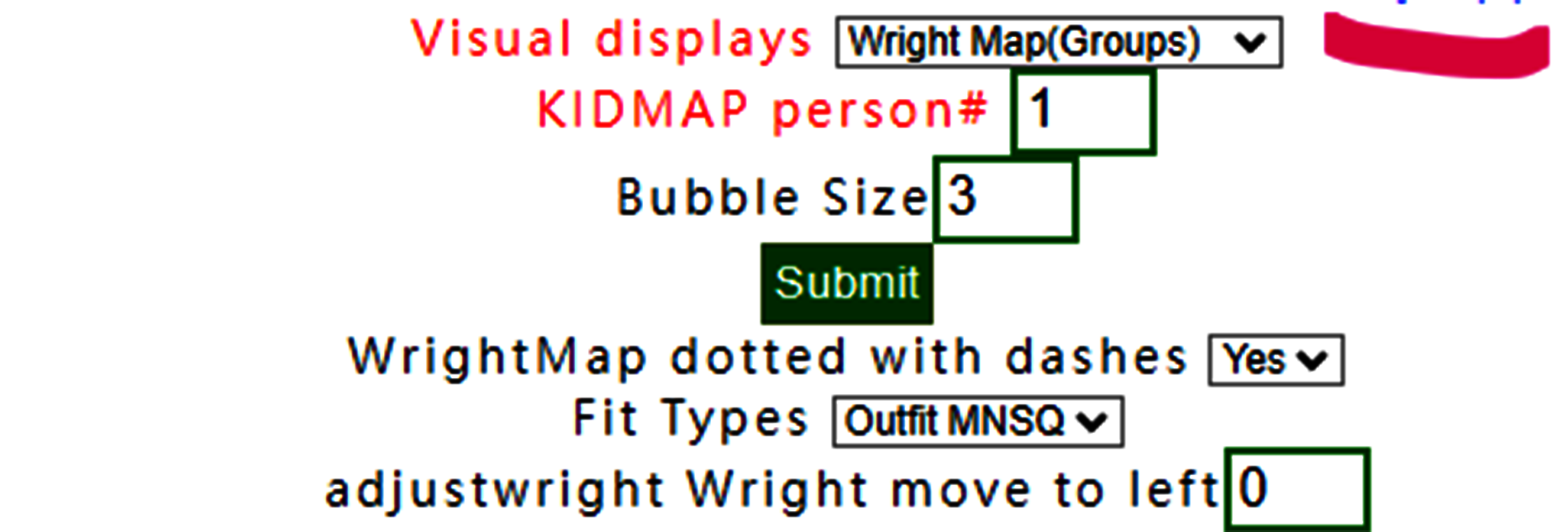## Wright Map with groups selected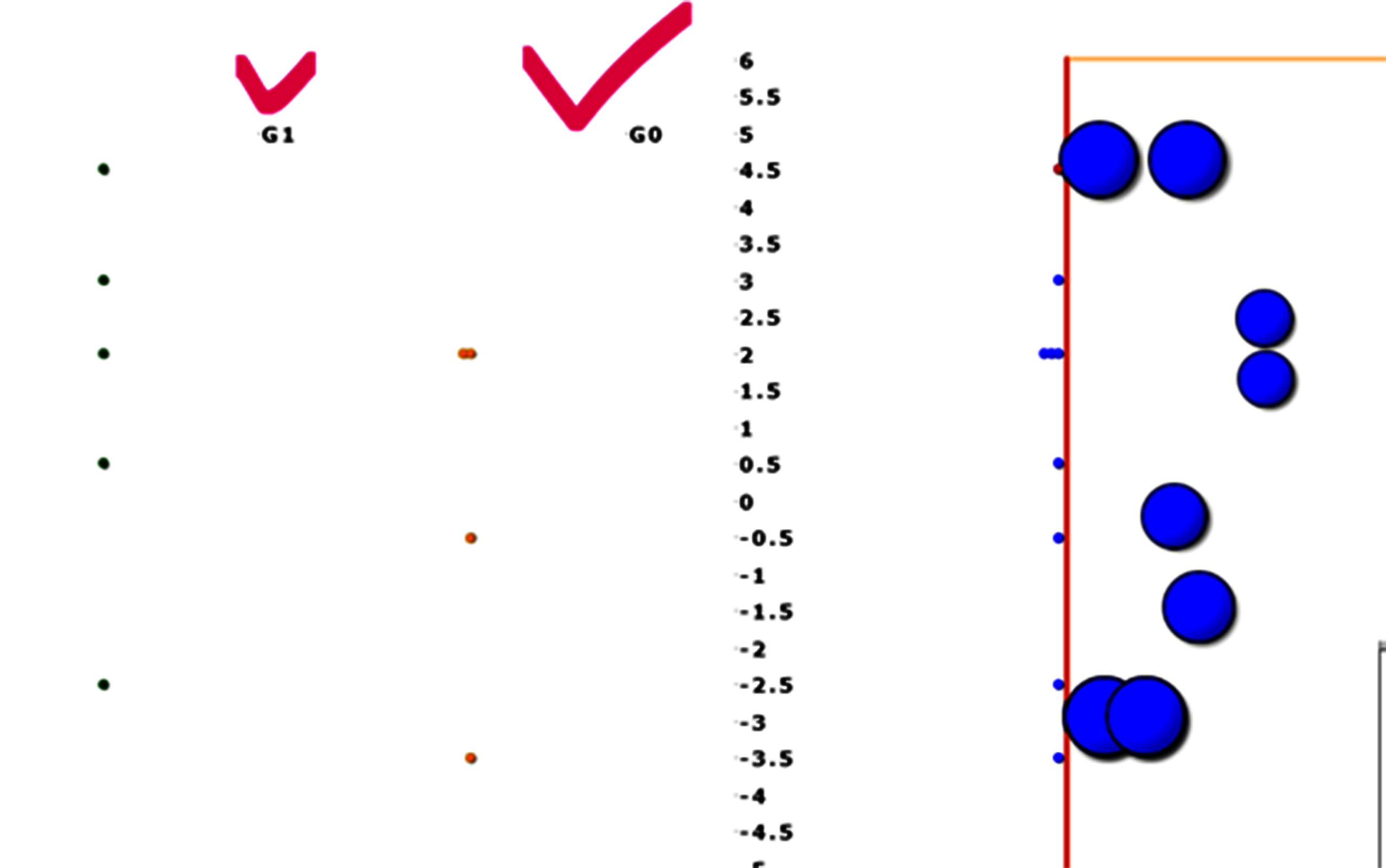## 7.KIDMAP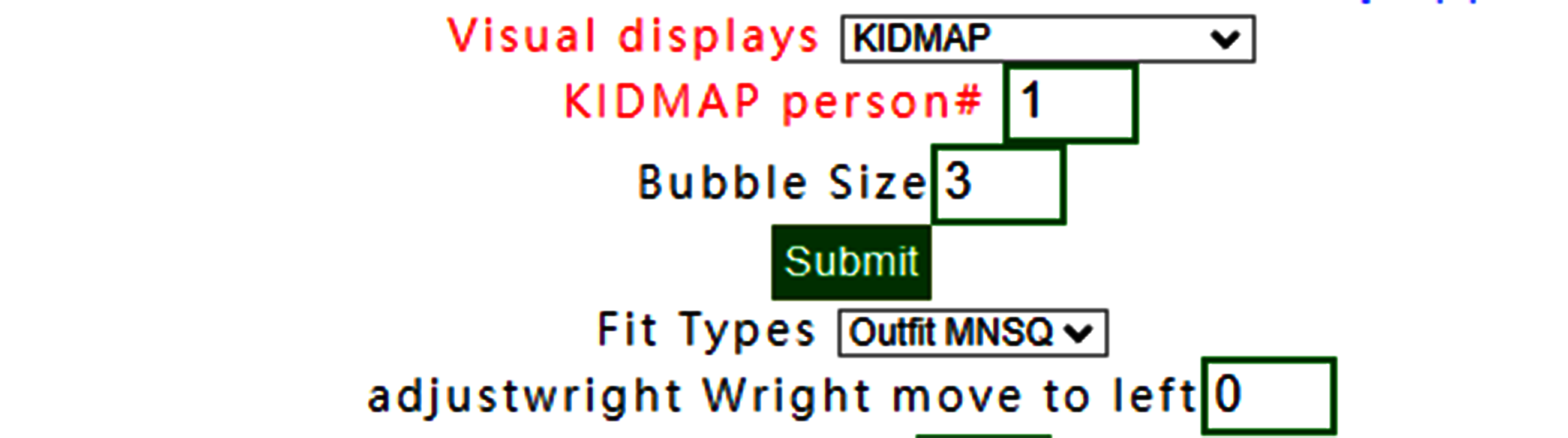## To select person number.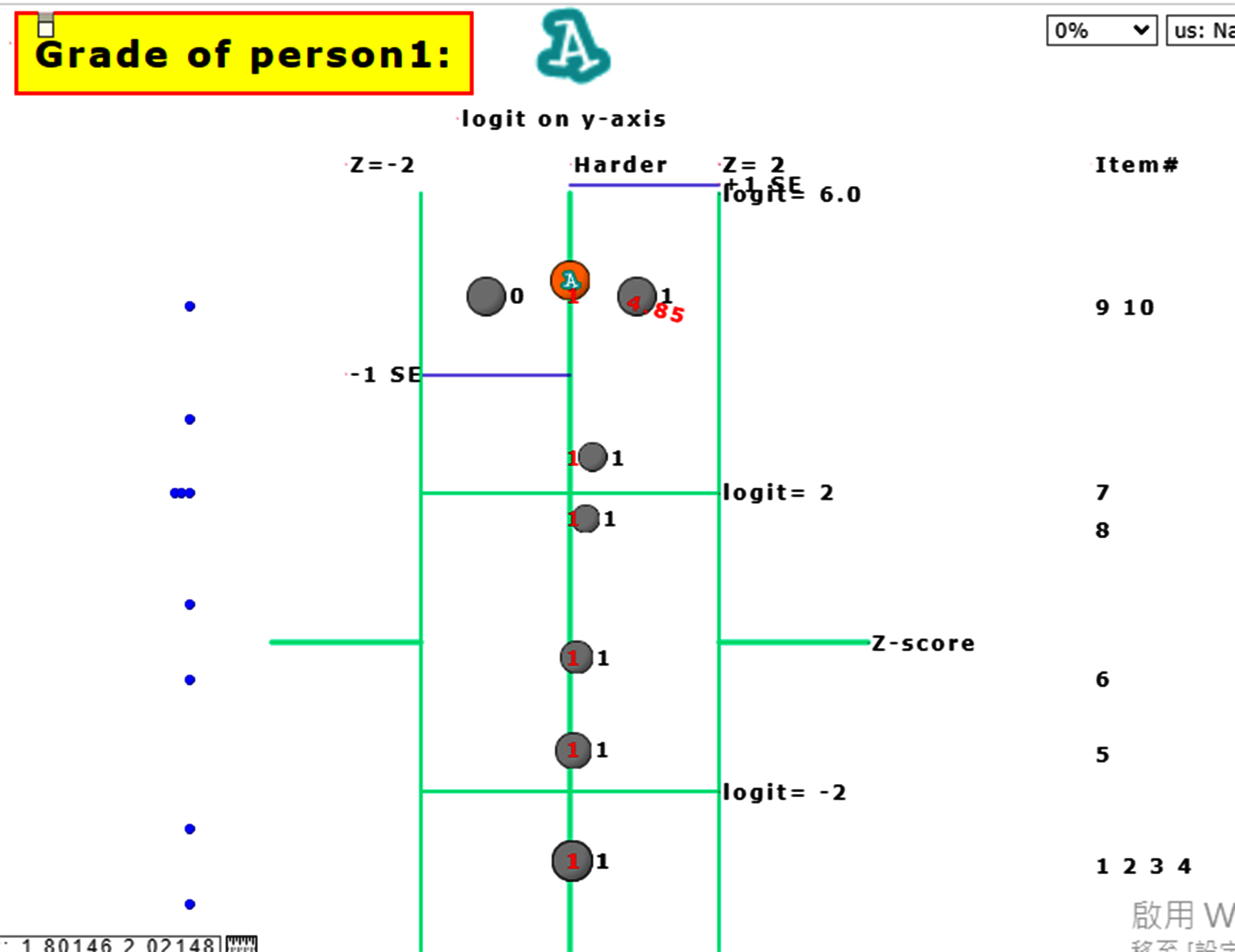## The one selected appears with a KIDMAP.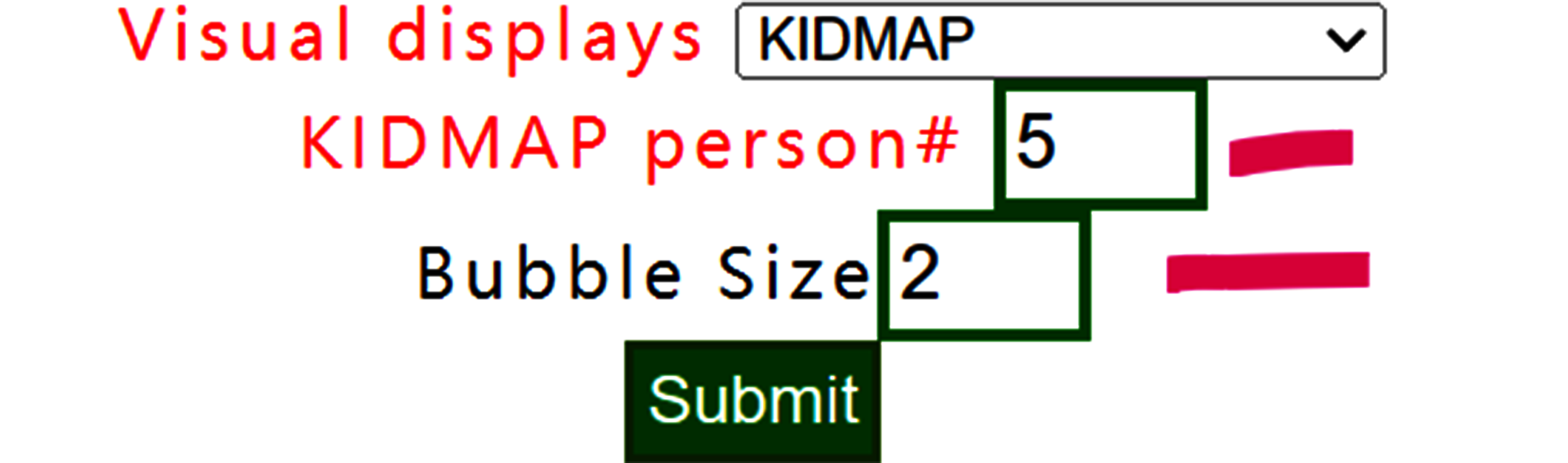## Another person is selected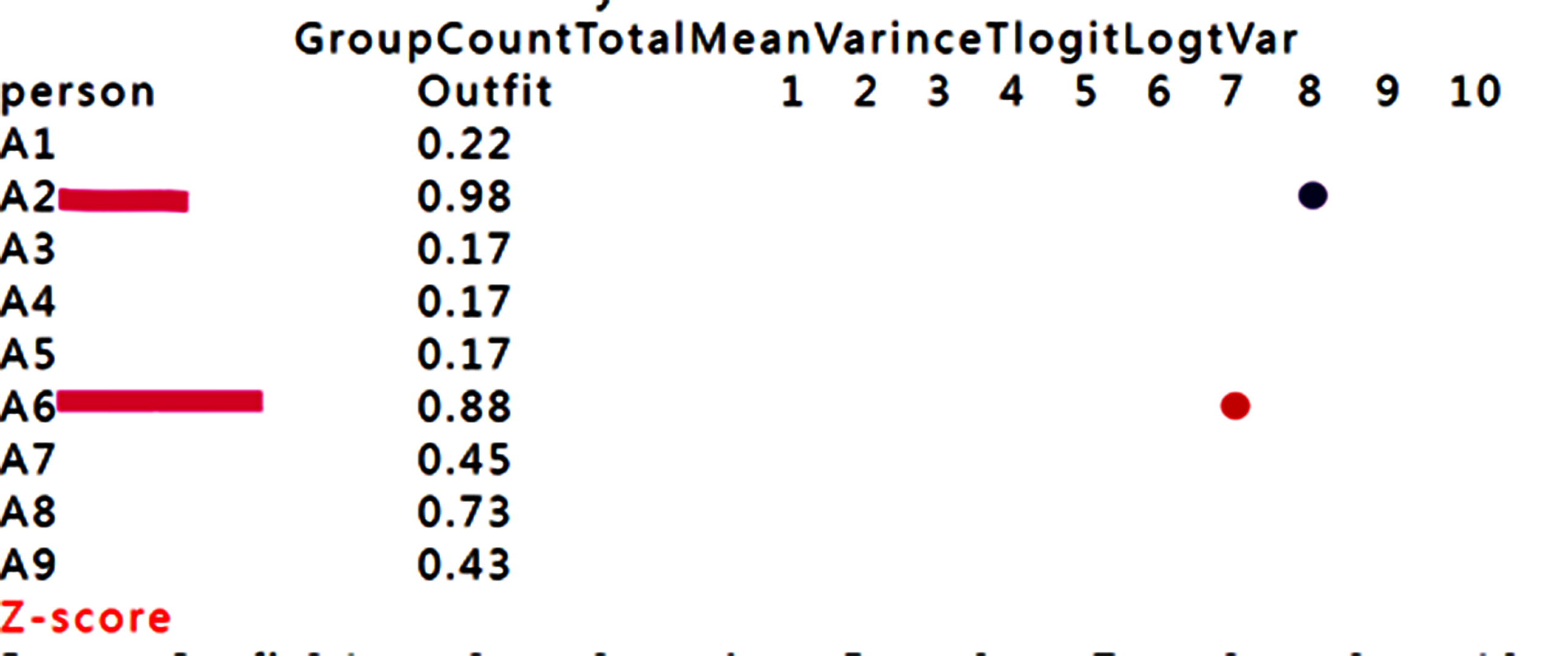## Based on the person measure and fit statistics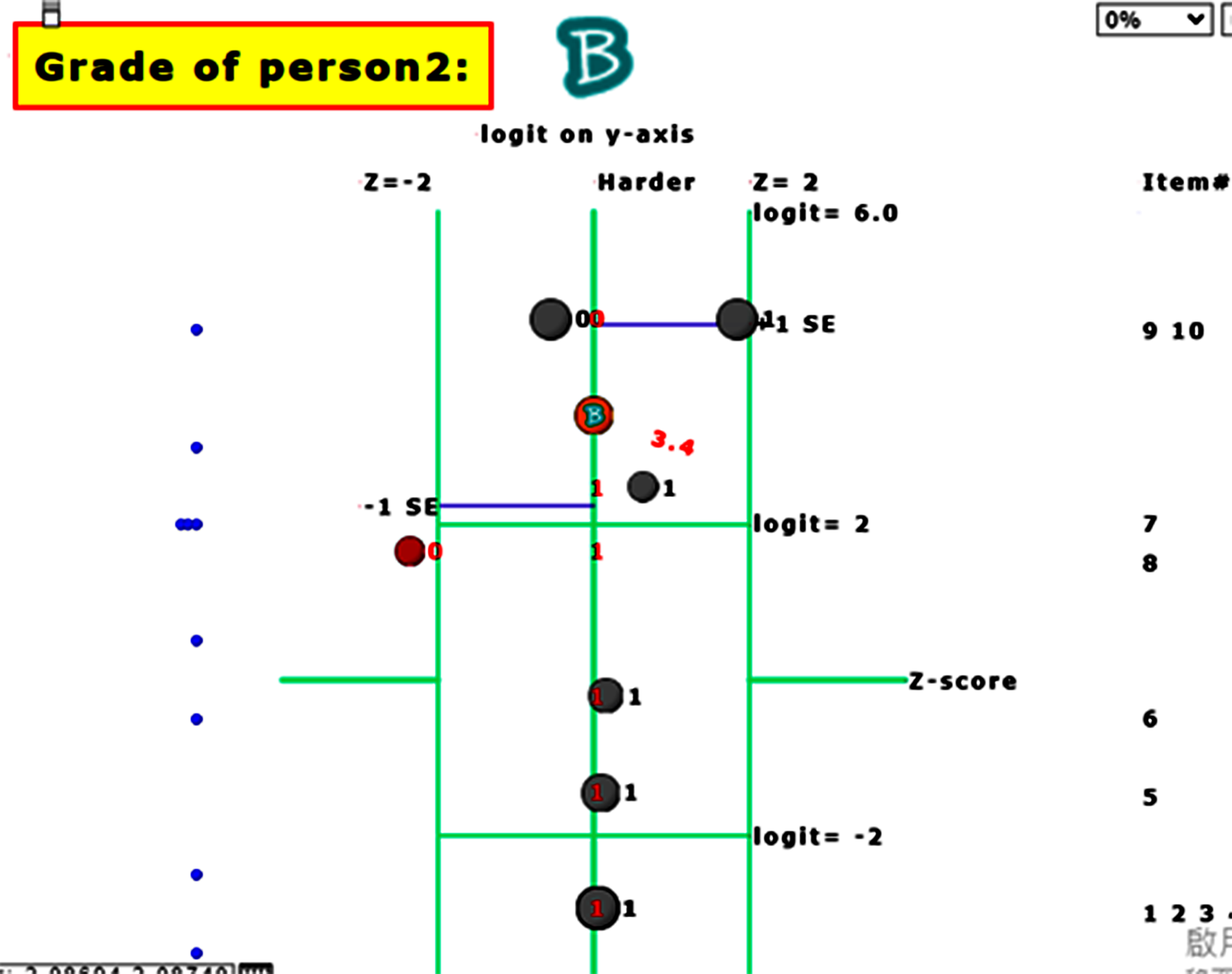## The one with one item unexpected is found using KIDMAP For person 2, we can see that item 8 in red bubble is unexpected(Zscore <-2.0) due to the easy item with incorrectness at the left-bottom side.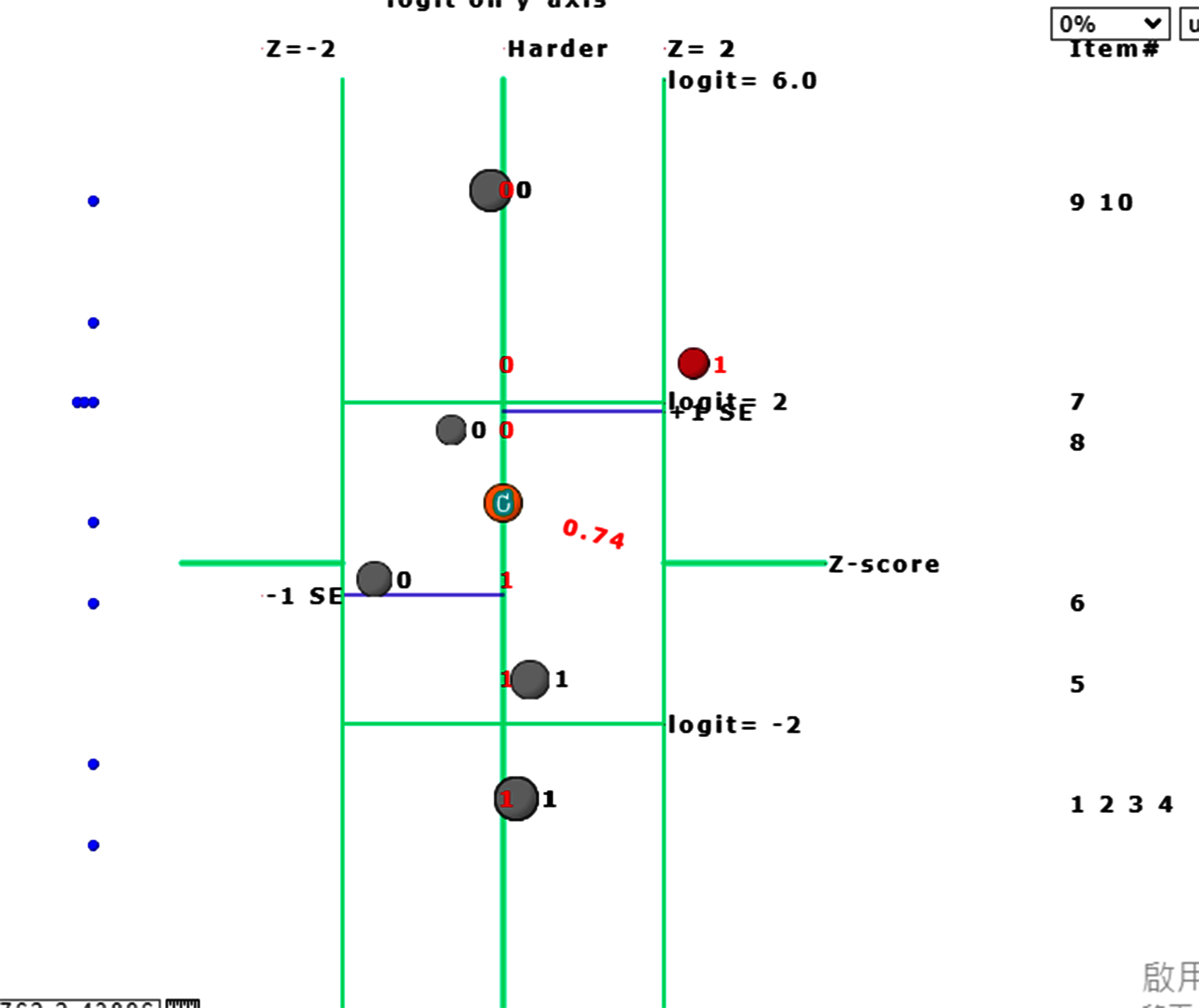## Another one with one item unexpected is found using KIDMAP. For person 6, we can see that item 7 in red is harder but correct in the right-top side(Zscore>2.0)## 8.ICC_cat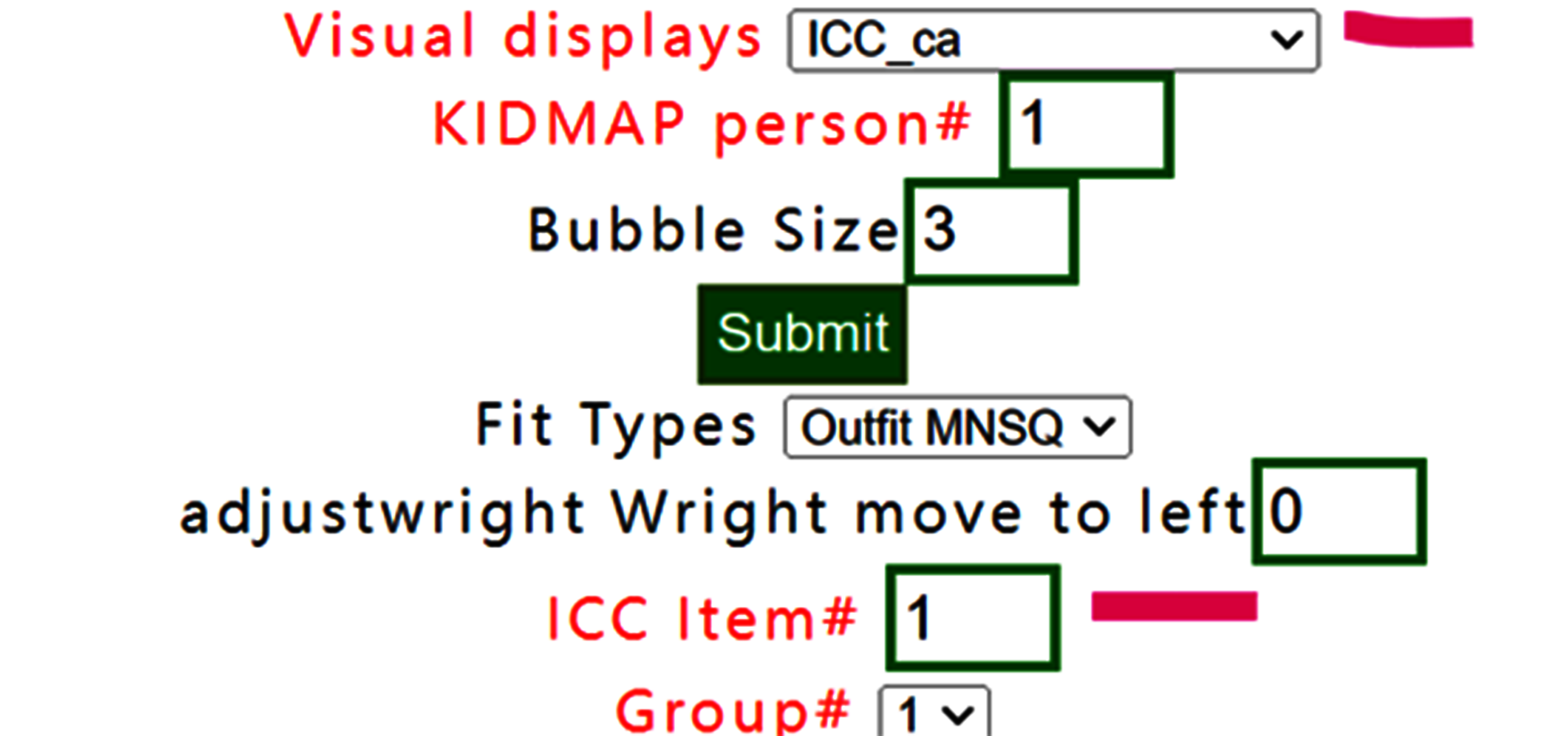## The CATEGORY PROBABILITIES: MODES - Andrich thresholds at intersections with ICC shows item# 1 for all persons below: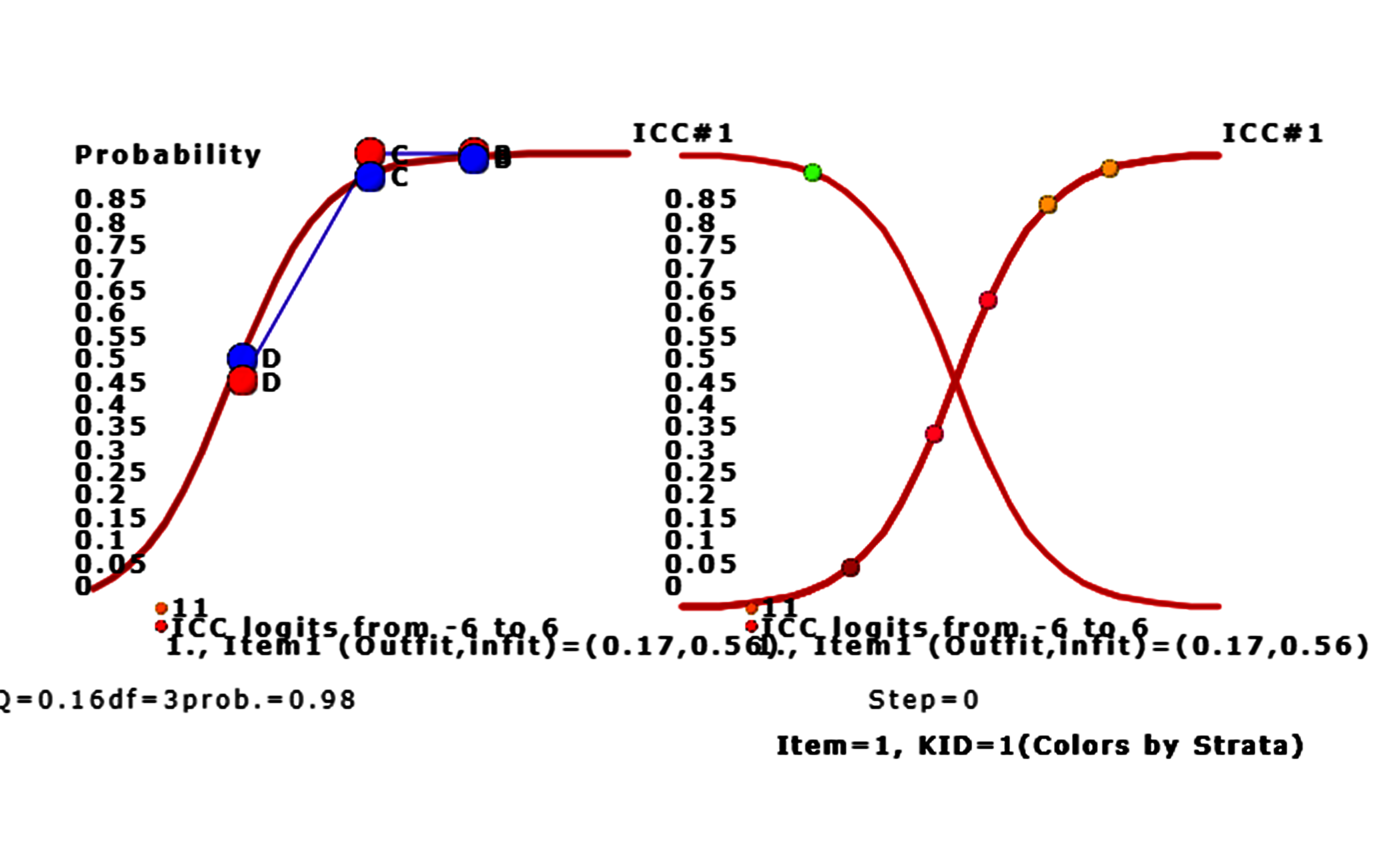## We can see that only person 9 answers tem 1 incorrectly. Other persons answer item 1 with correctness on the success ogive cure for left-bottom to right-top corner. Persons with higher ability are on the right-hand side. The bubble denoted by the vertical probability corresponds to the person ability. Moving to the left on Google Maps, the ICC of item 1 is shown above. The red bubbles denote the observed responses by persons and the blue ones represent the expected responses by persons. The ChSQ statistics shown below, similar to the overall fit selected in the menu at the beginning.## Bubbles are colored by groups. We can see that only person 9 answers item 1 incorrectly. Other persons answer the item 1 with correctness on the success ogive cure for left-bottom to right-top corner. Persons with higher ability are on the right-hand side. The bubble denoted by the vertical probability corresponds to the person ability. To add lines linked to observed bubbles in ICC## The lines have been linked for observed responses of groups.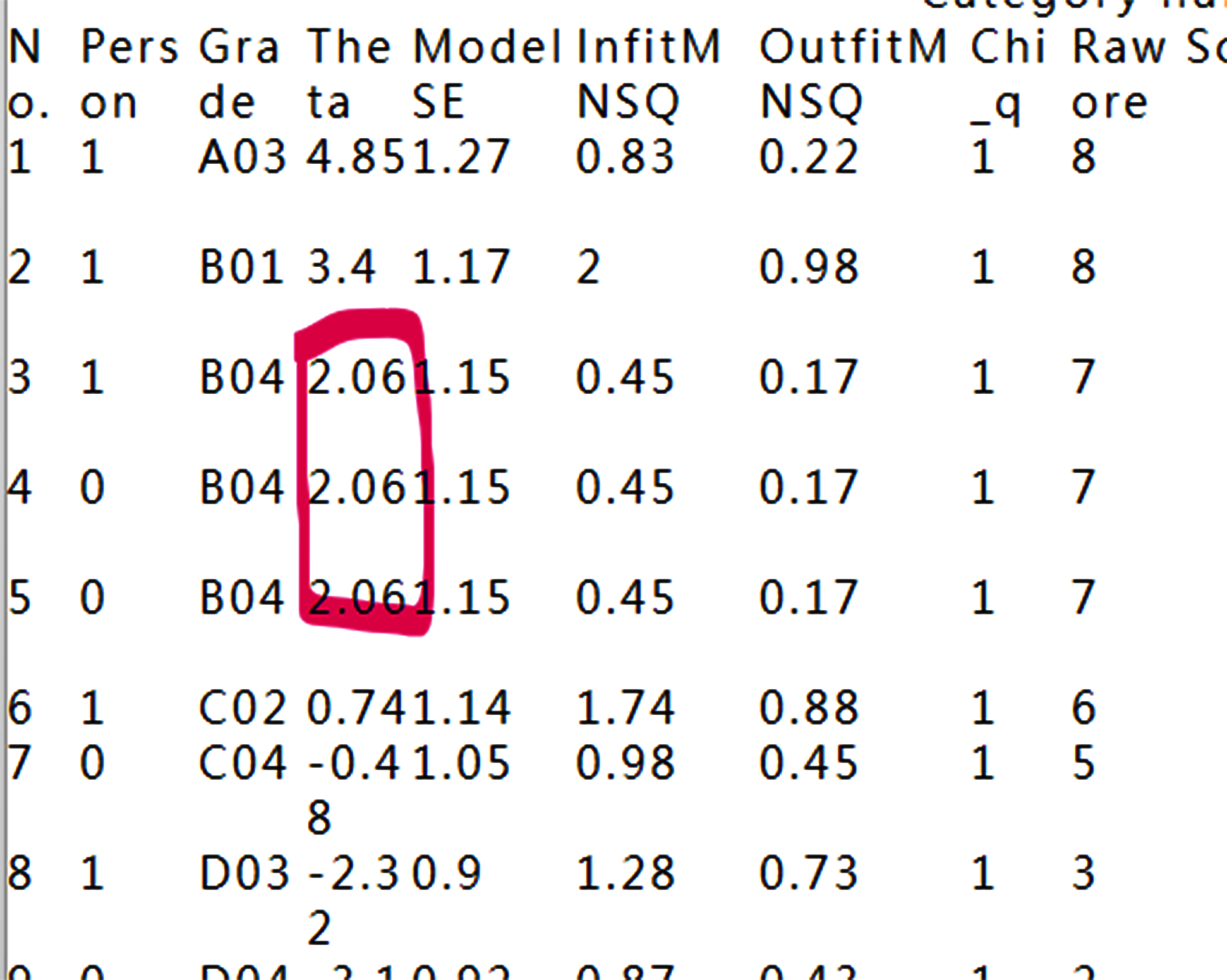## 9.Person fit plot(Outfit)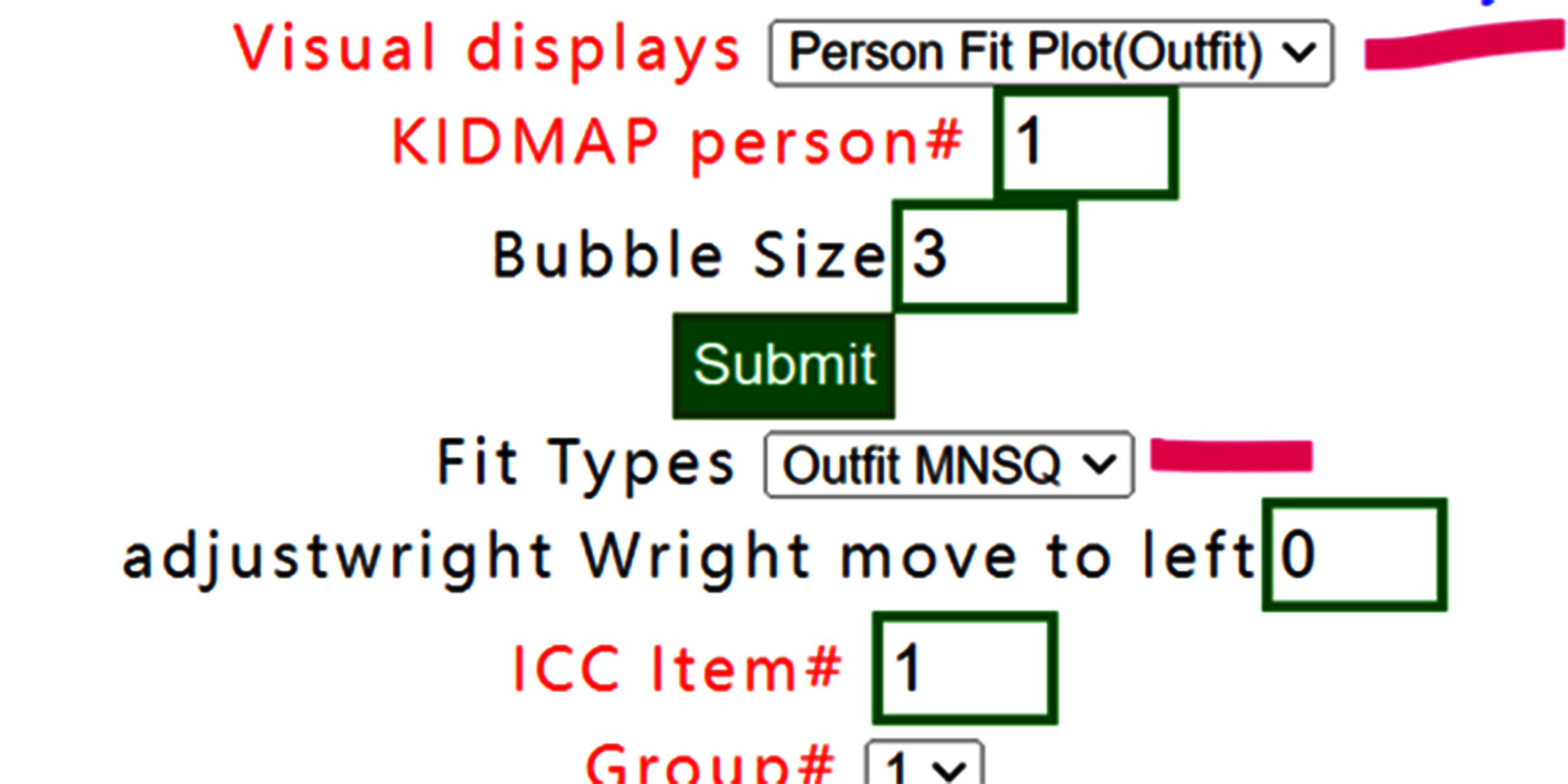## Select person fit plot with outfit MNSQ,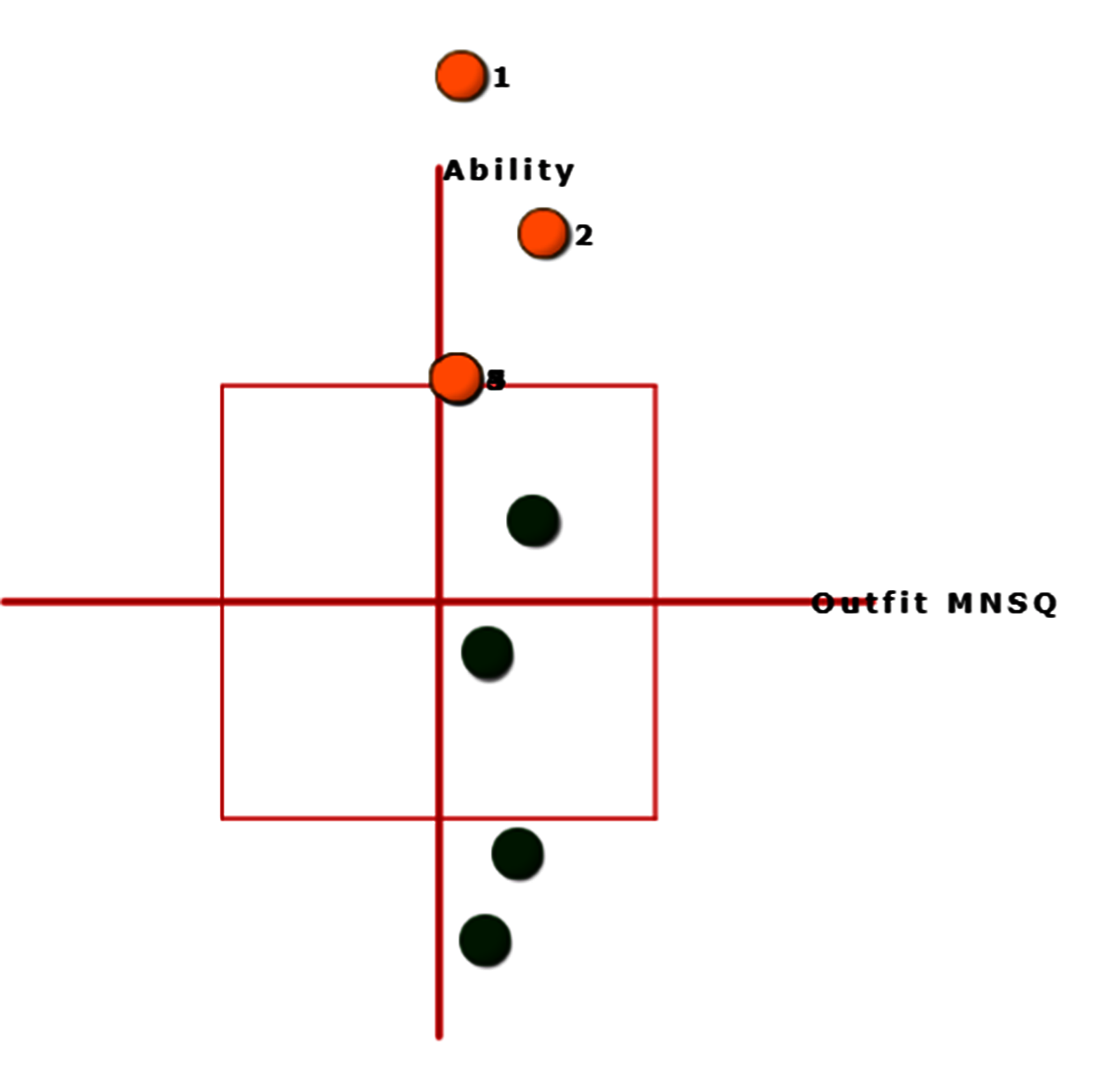## 10.Person fit plot(Infit)## If Infit MNSQ is selected, the person fit plot is shown below: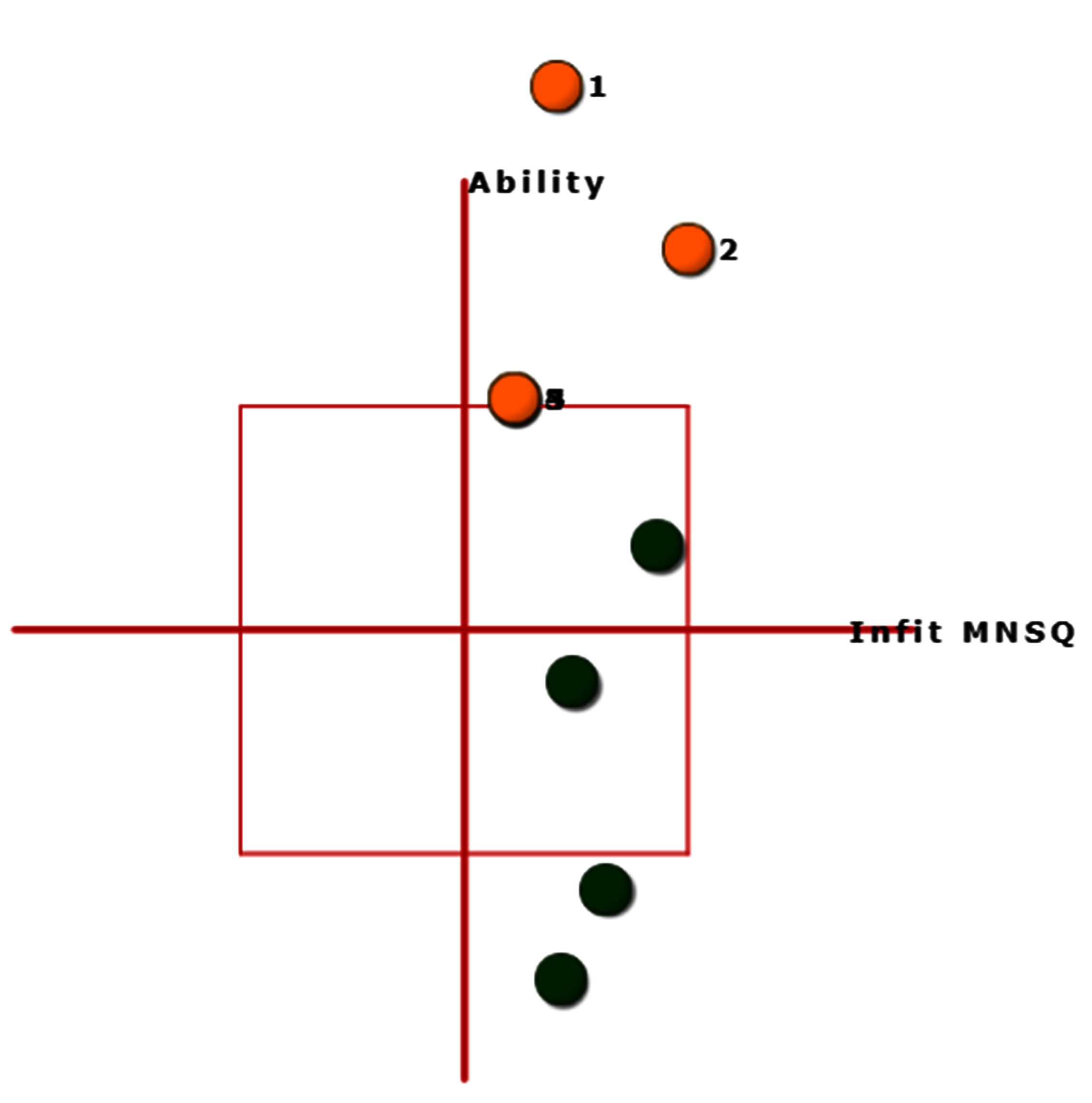## 11.Measure/Outfit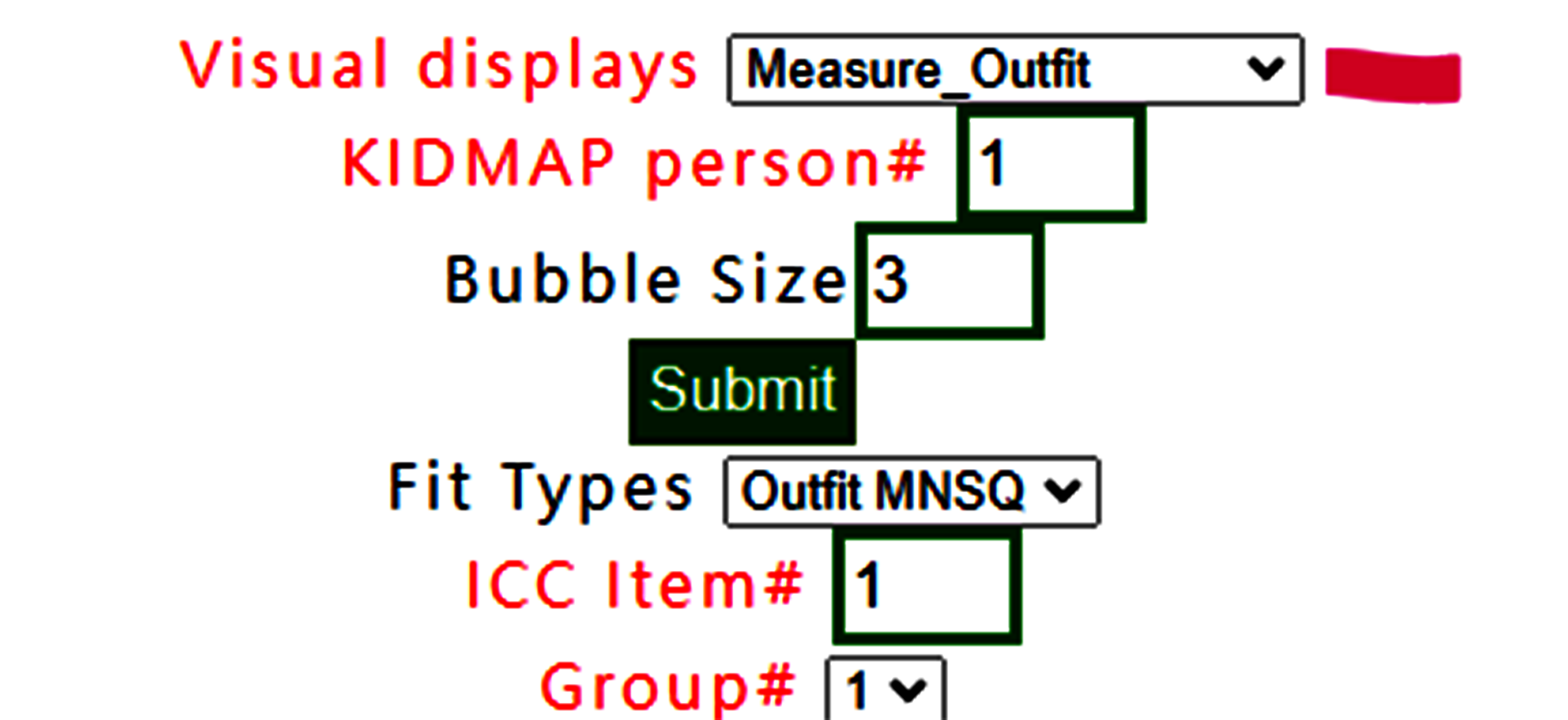## Measure Outfit is selected.## The buffered form is generated. After confirming it, the plot is shown as below: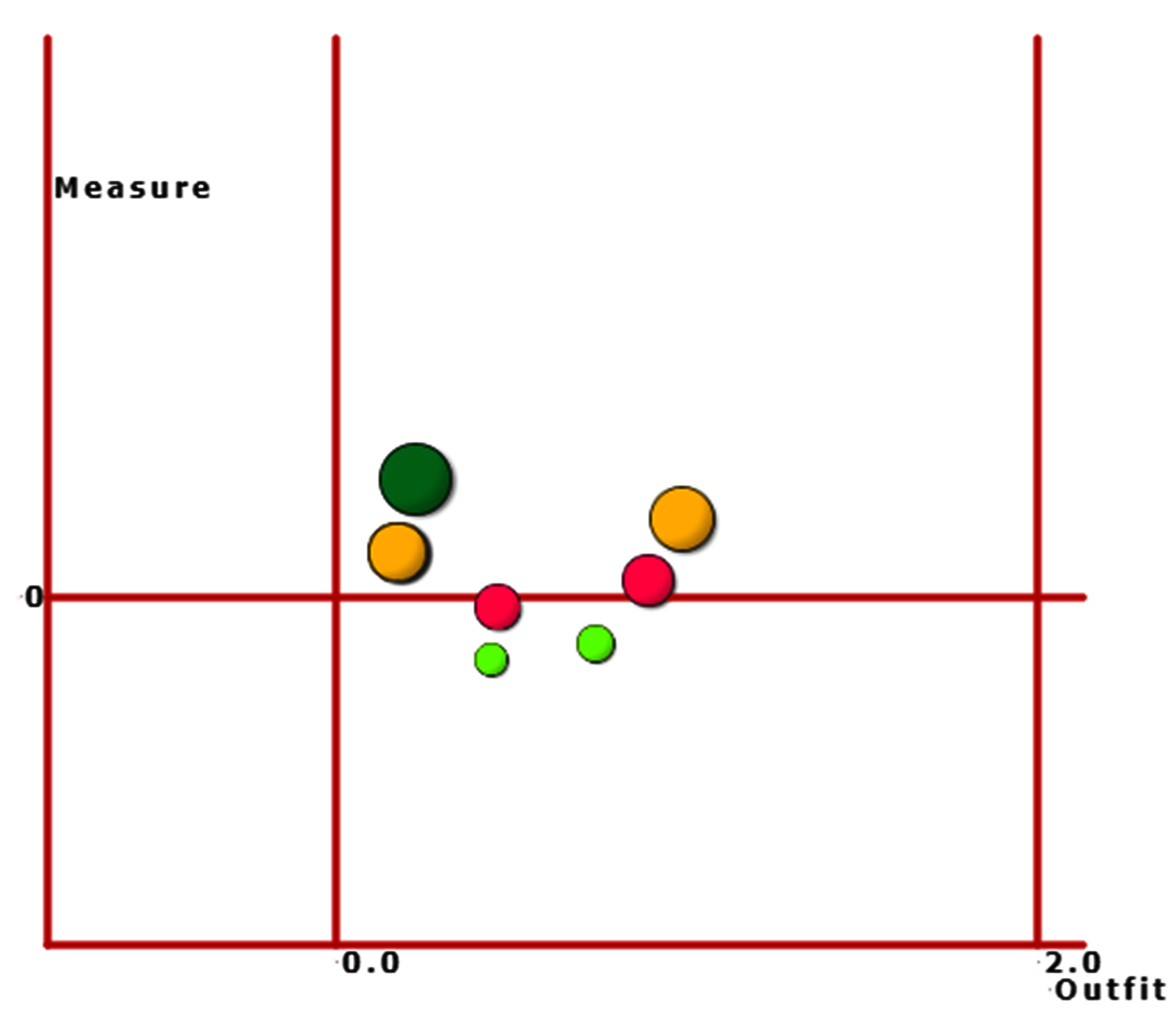## 12.Measure/Infit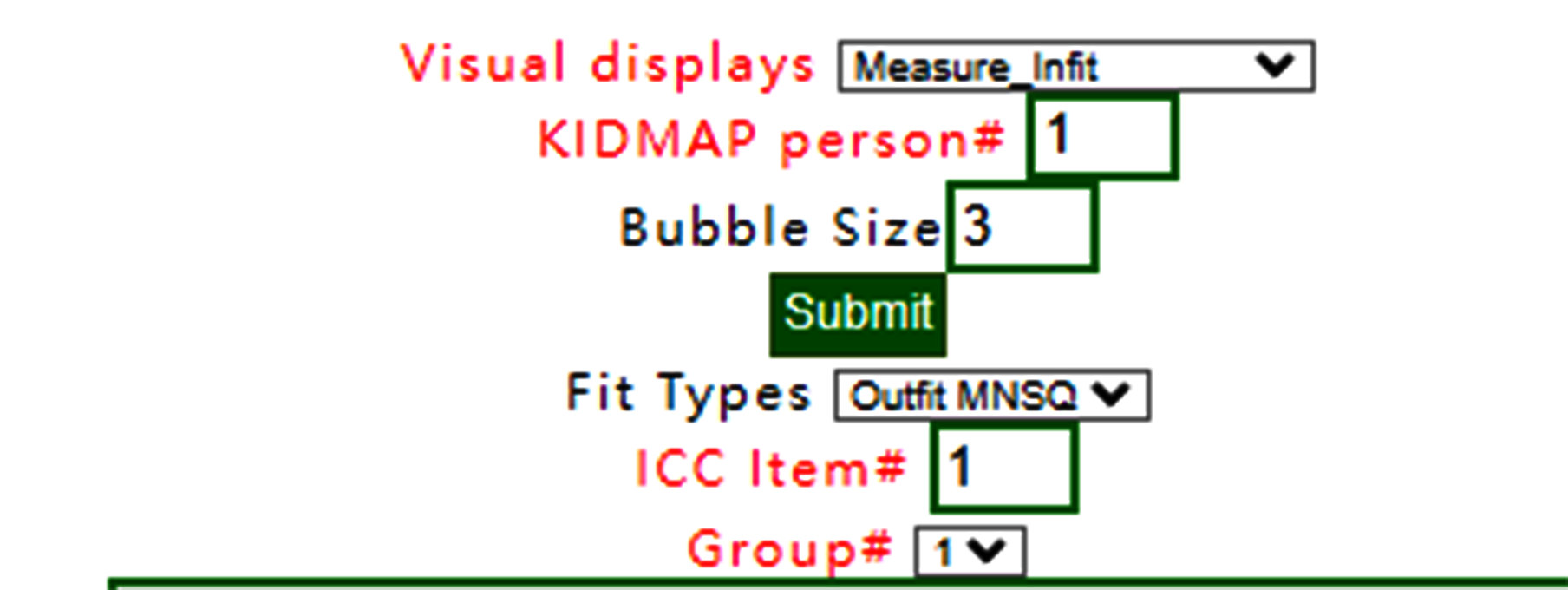## Measure Infit is selected.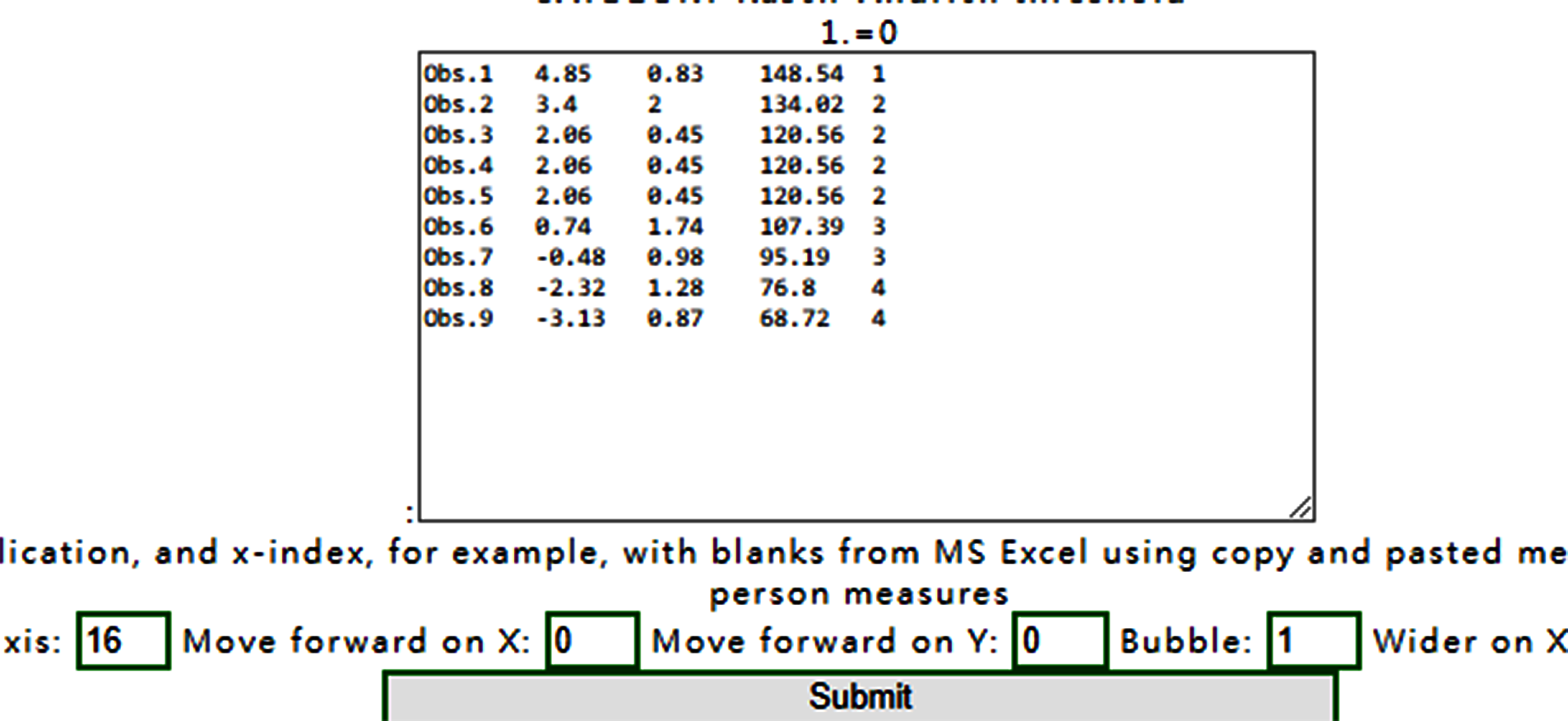## The buffered form is generated. After confirming it, the plot is shown as below:## 13.Rawscore/Measure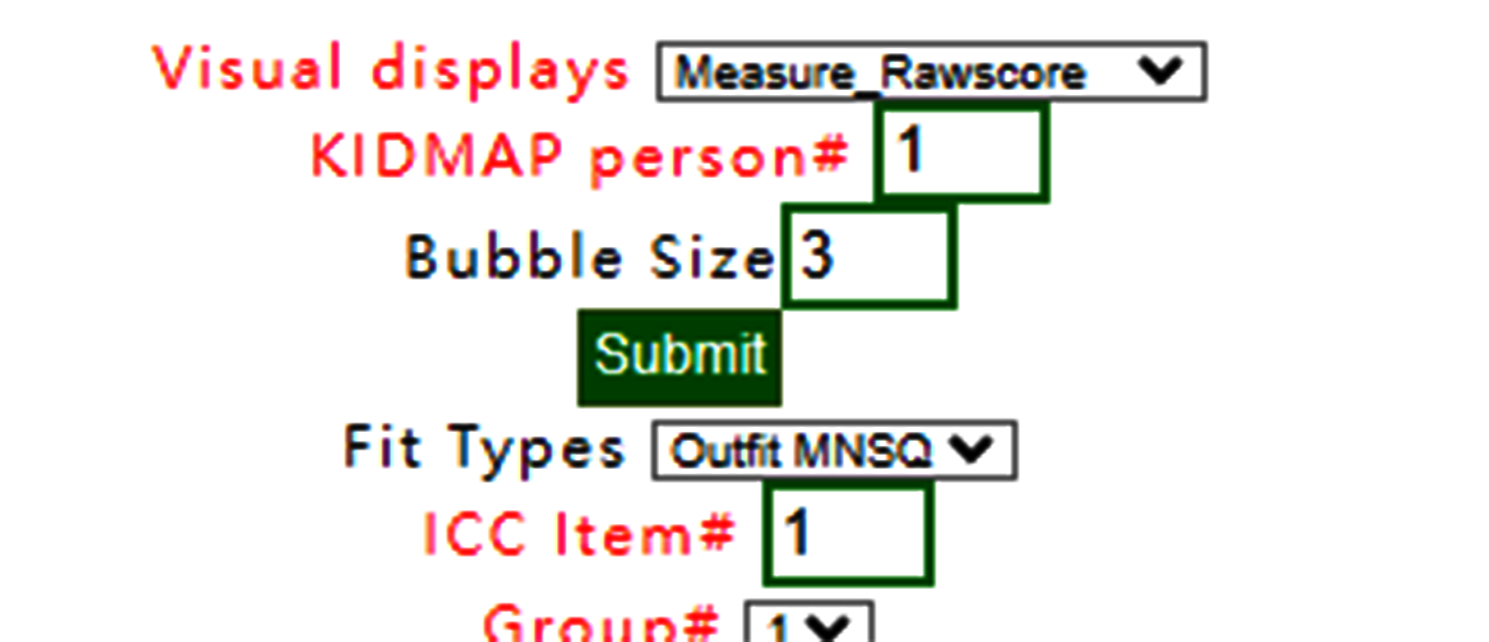## When Rawscore/measure plot is selected using Kano diagram, the confirmation is shown below:## Confirm it and show the Kano diagram below: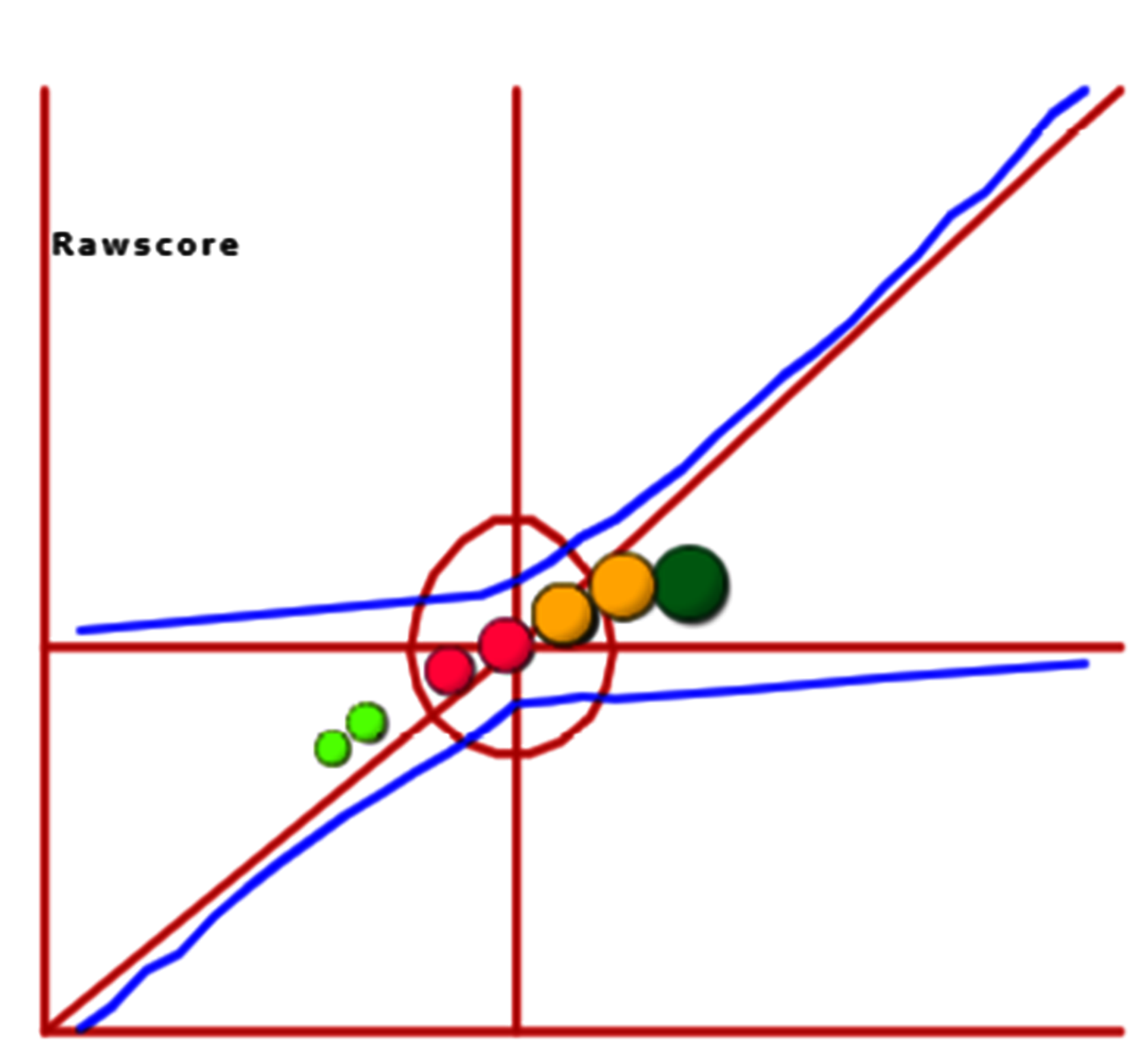## 14.Simulation data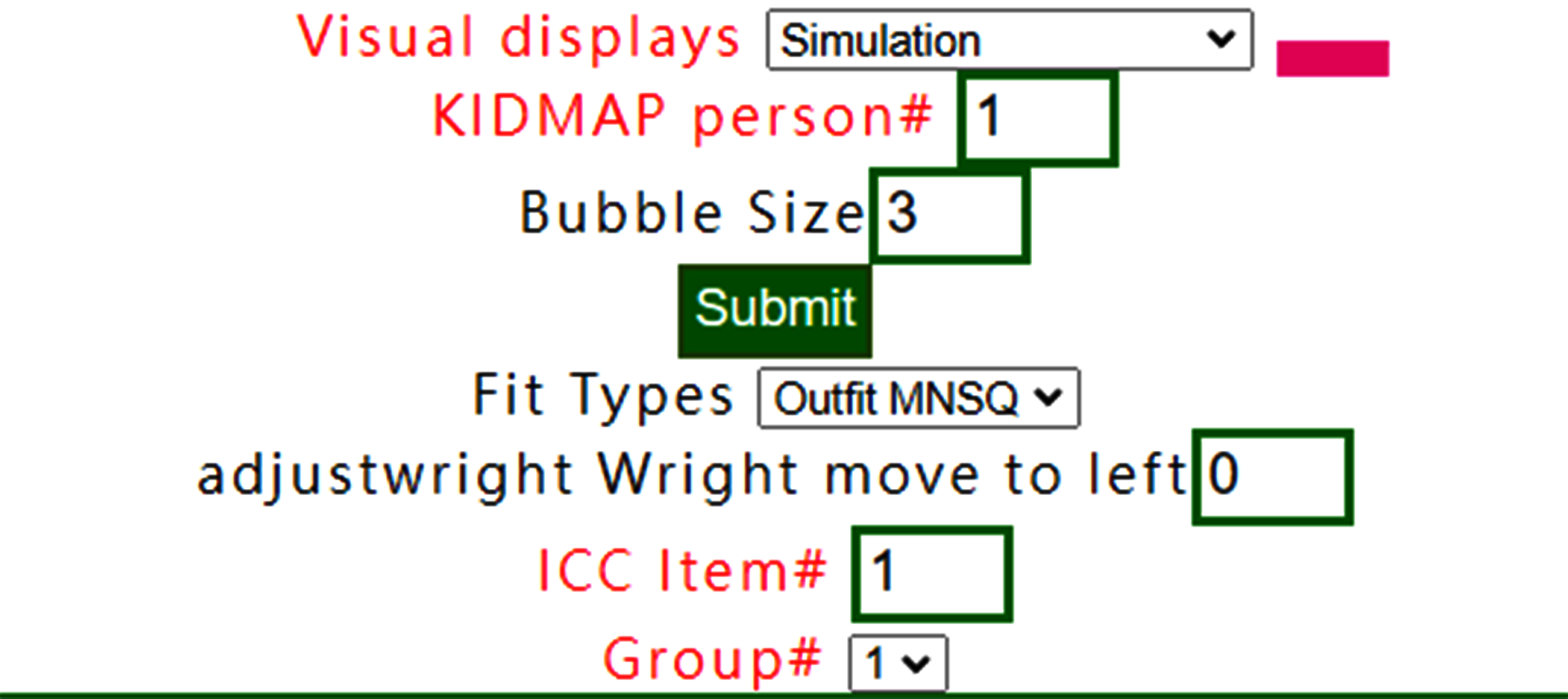## The simulation data can be generated based on the reference at https://www.rasch.org/rmt/rmt213a.htm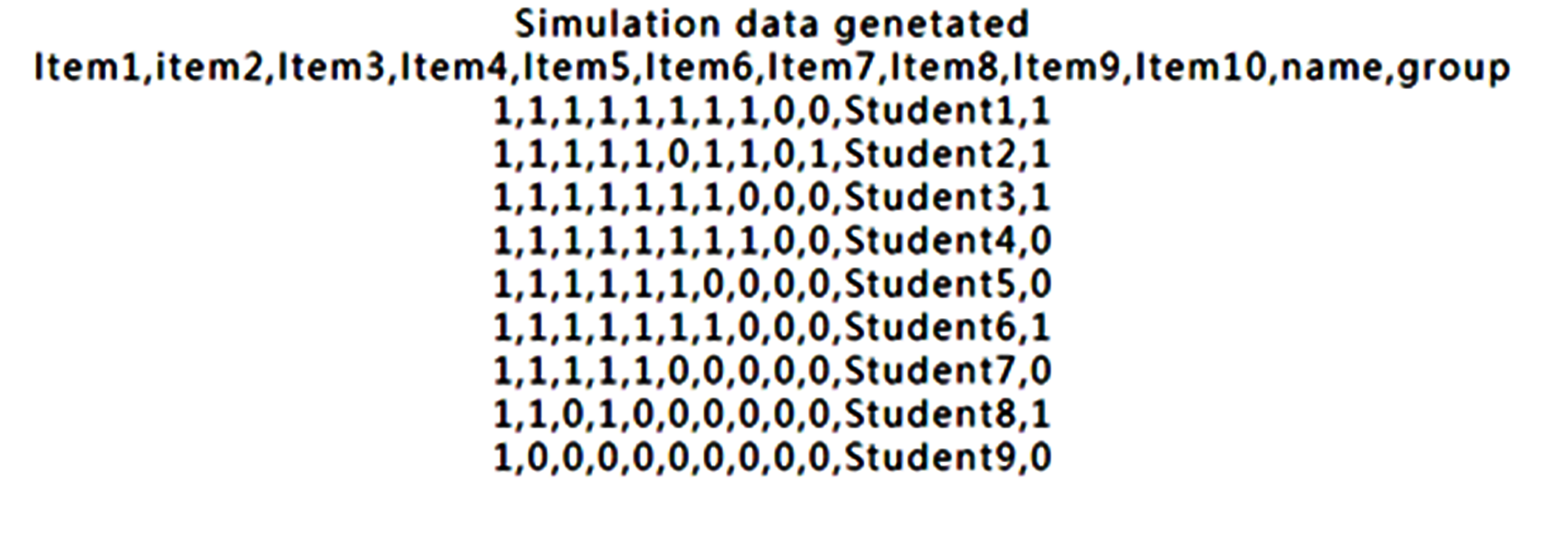## The result below the website is the simulation data. We copy those data onto RaschOnline.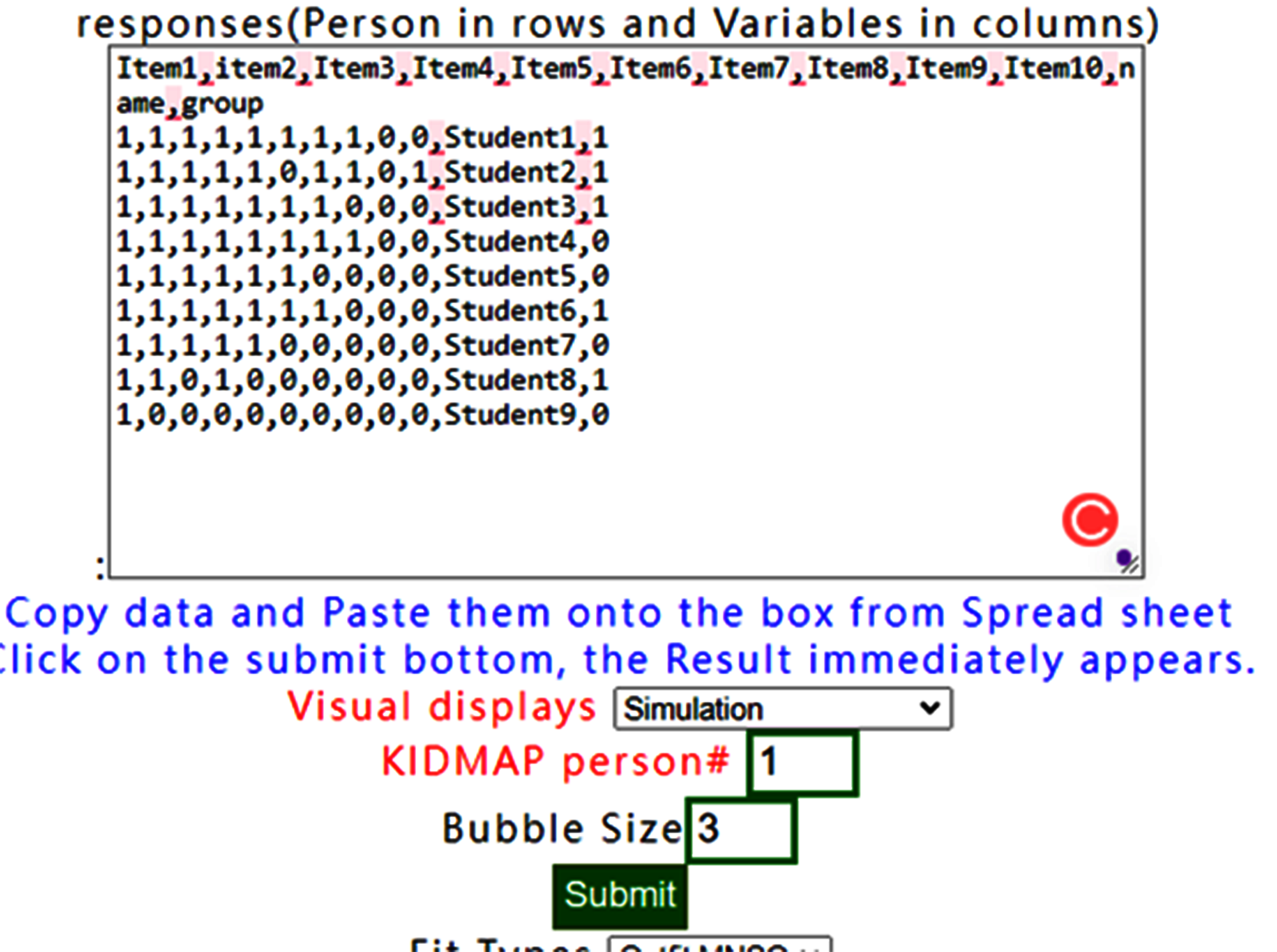## We can see that all person measures are along with raw scores on the one-dimensional part in the diagram. Like this one above. If the visual display with None is selected, the results show all items fit to Rasch model when observing all infit/outfit MNSQ smaller than those in the original dataset.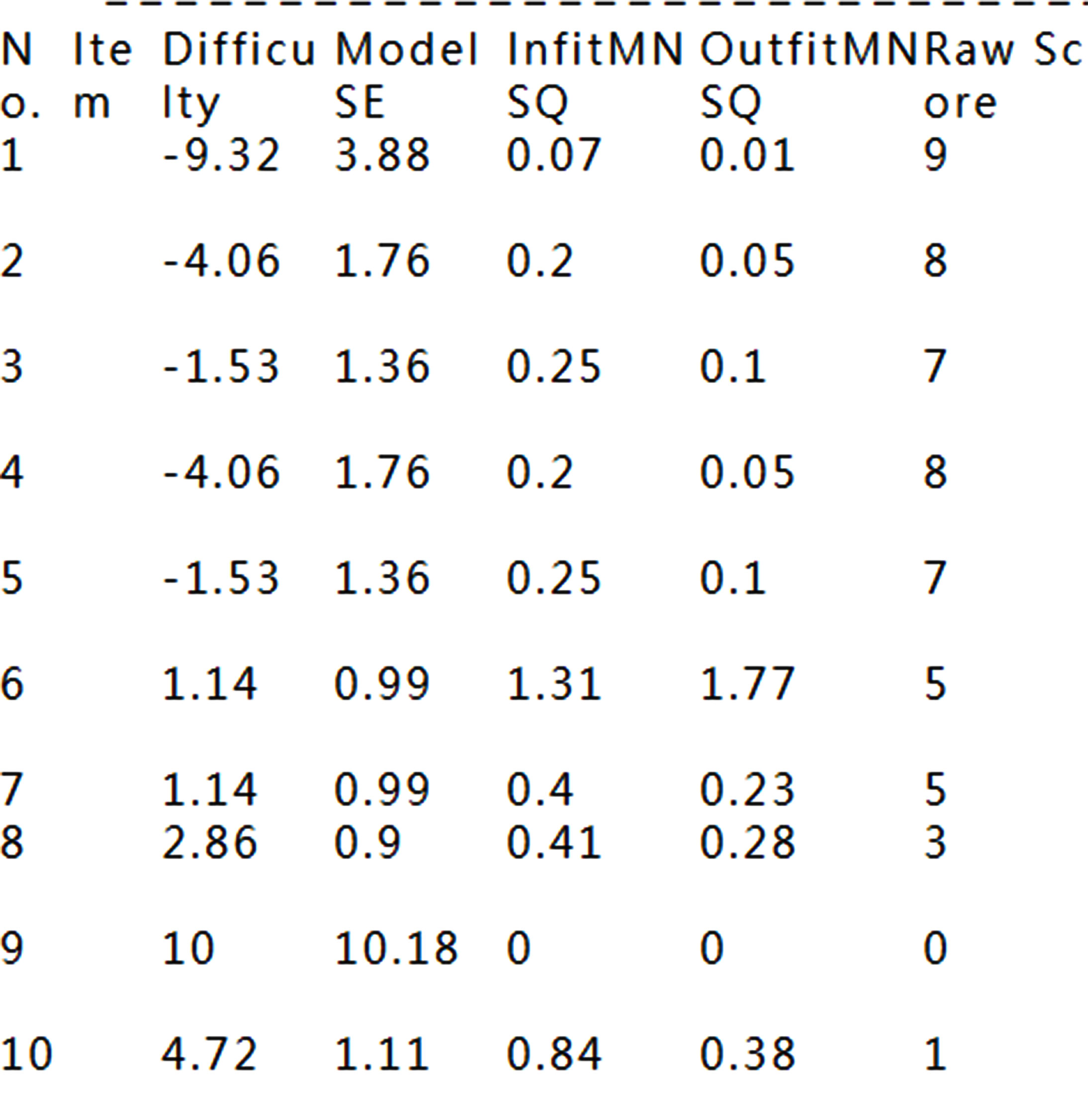## See how well items fitting to the Rasch model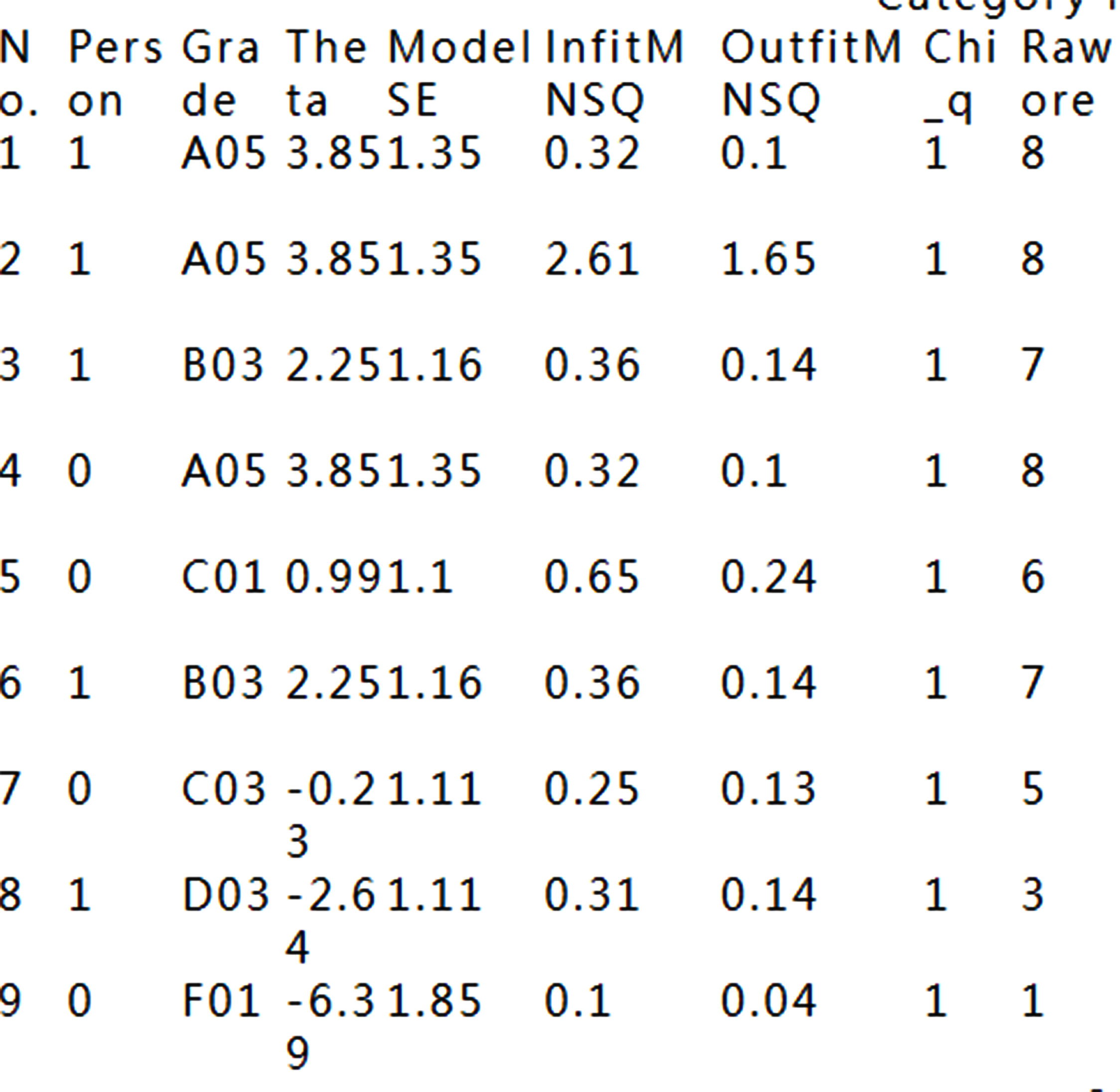## That is because the data fit Rasch model fairly well.## 15.DIF:Table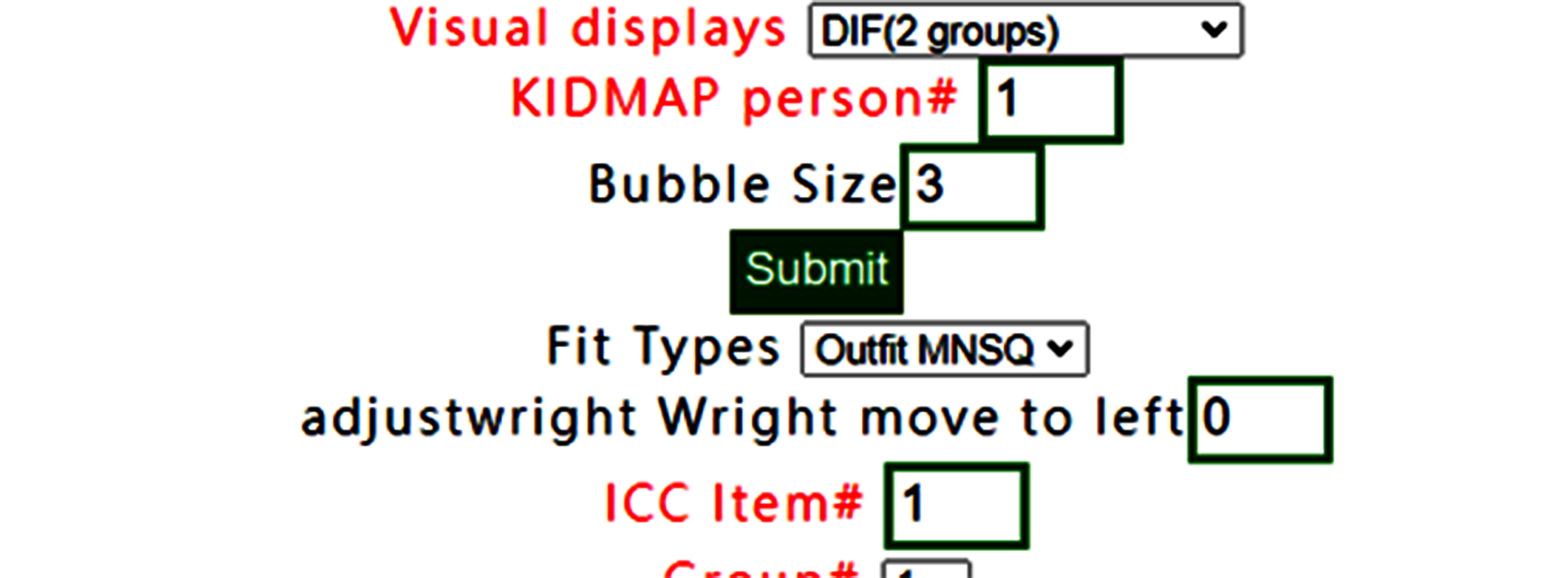## DIF(Table) has been selected(note. only for two group, 0 and 1 in group definition in RaschOnline).## 16.DIF:Graph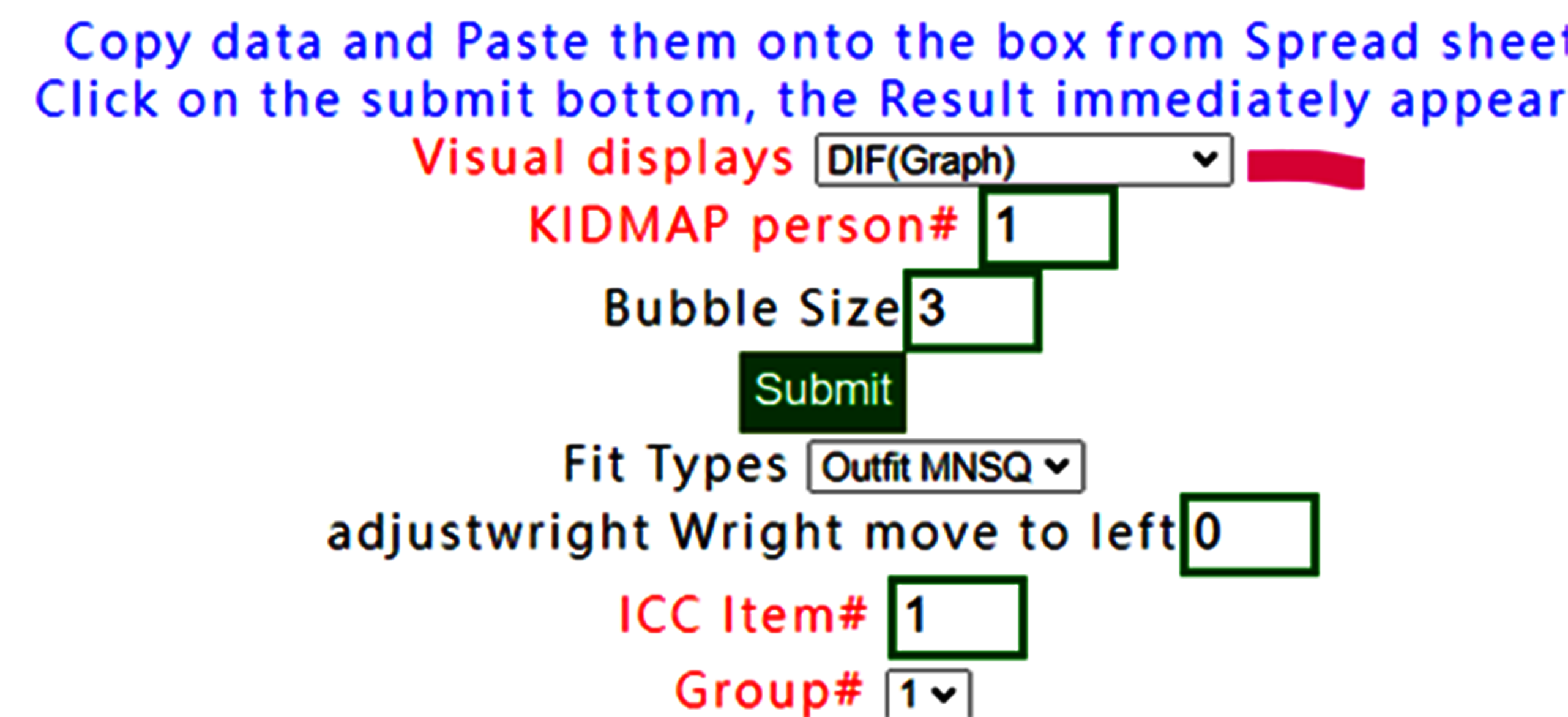## If DIF graph is selected, the graph using forest plot appears immediately on Google Maps after confirming the results below.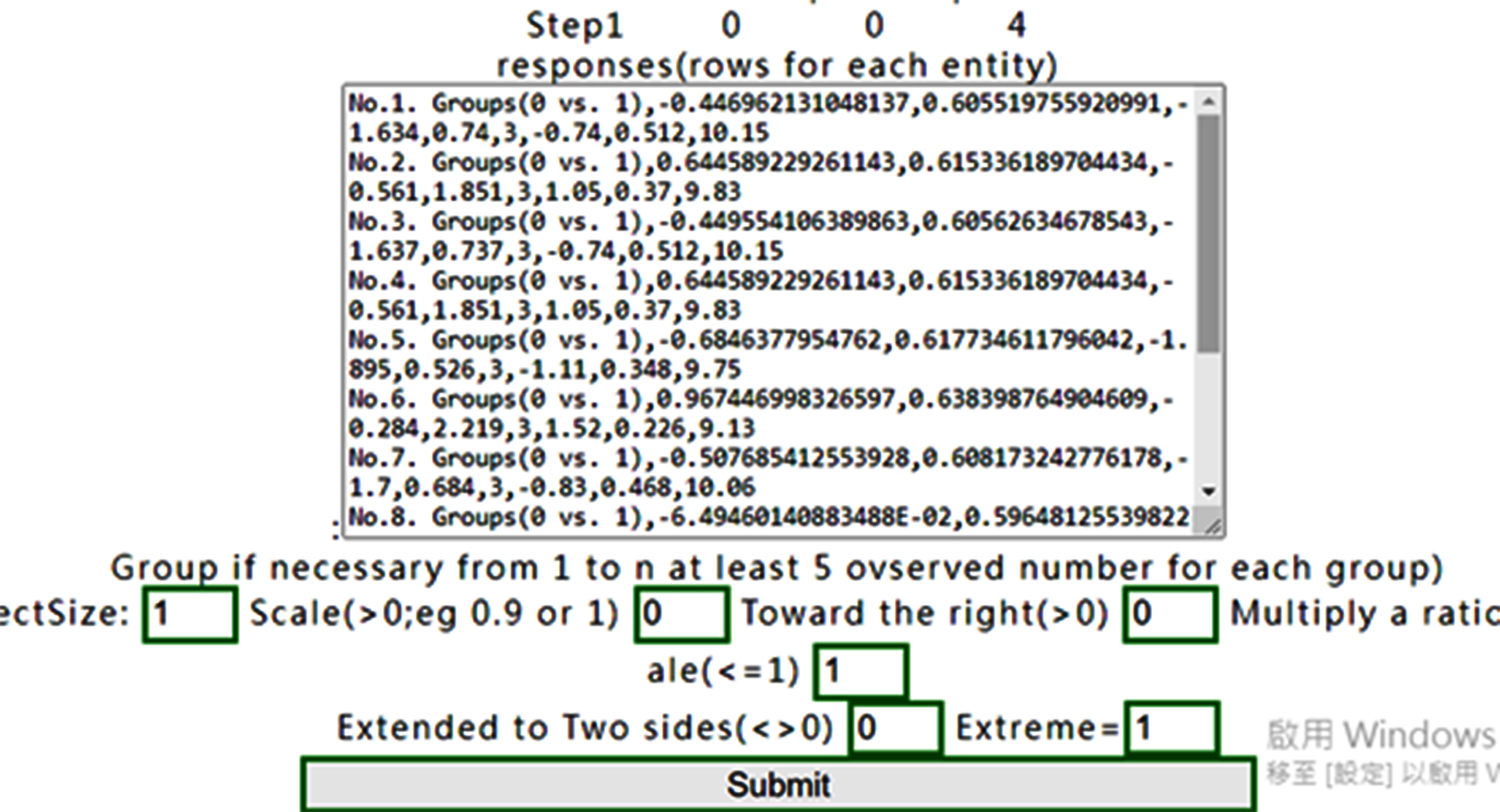## Confirm the contents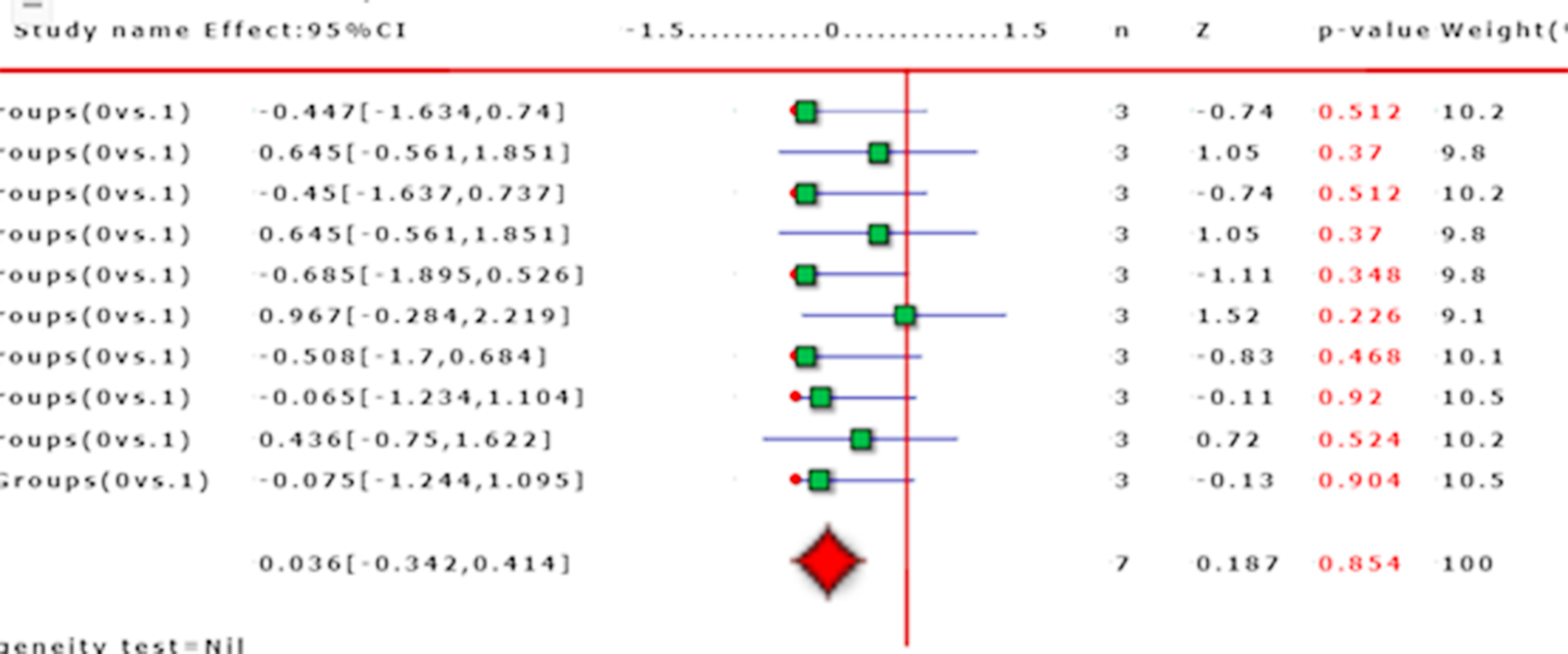## preparedness of Copy&paste data including head titles and last two with "name" and "group"## Paste data into the frame and then click on the submit bottom if no others selections necessary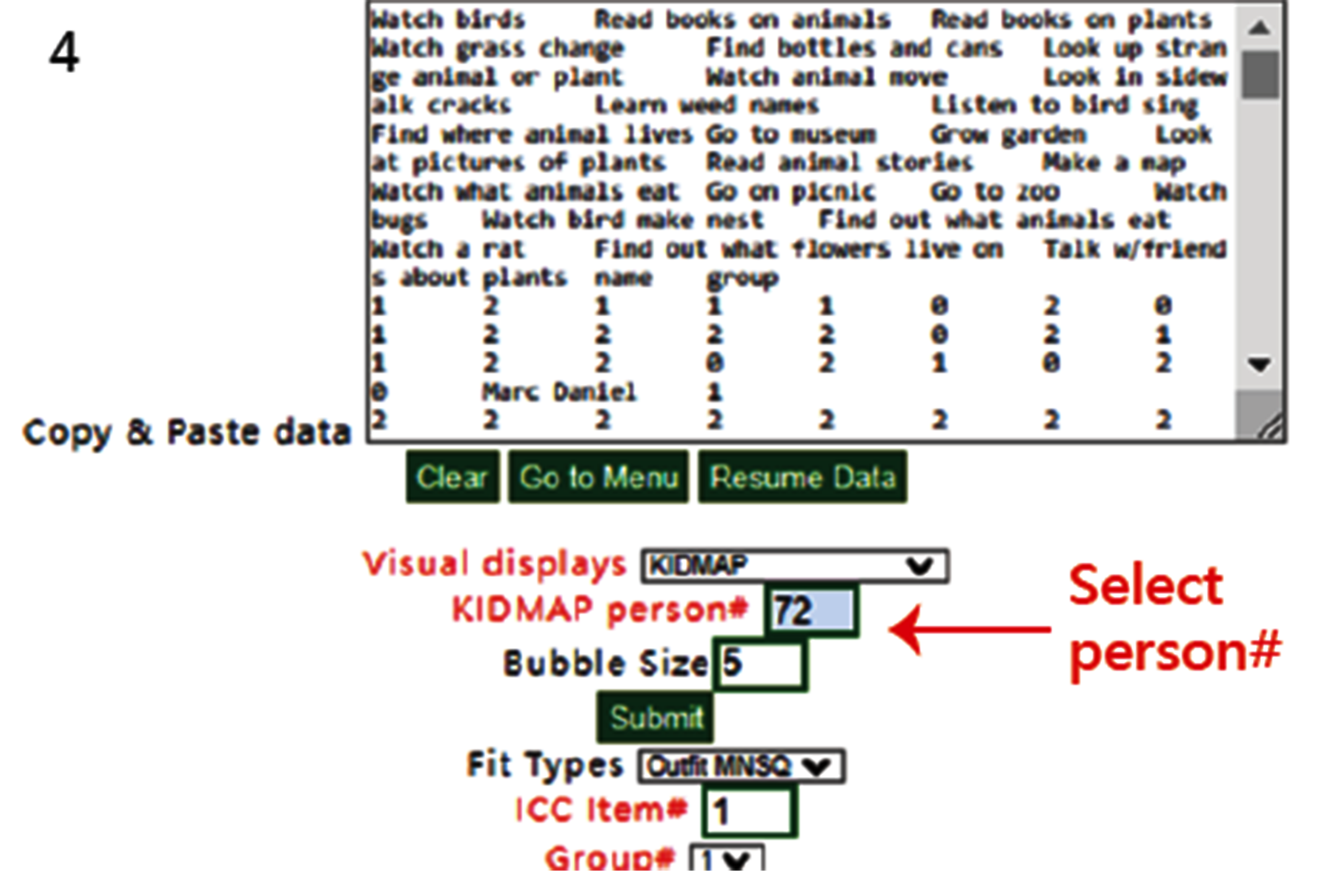## Results displayed